# Maharashtra State HSC Board Question Paper for Class 12th Maths 2018 In PDF

## MSBSHSE HSC Board 12th Class Question Paper Mathematics English Medium 2018 with Solutions – Free Download

Maharashtra State Board Class 12 maths 2018 question paper with solutions are available on this page, by BYJU’S, in downloadable pdf format and also in the text for the students to prepare well for the MSBSHSE exams. Maths Question Paper 2018 Class 12 is attached here for reference. Students are able to access all the Maharashtra HSC Board previous year maths question papers. By solving these papers, students can familiarise themselves with the question paper pattern and also the mark distribution for each of the chapters.

### MSBSHSE Class 12th Maths Question Paper With Solutions 2018

#### QUESTION PAPER CODE J – 265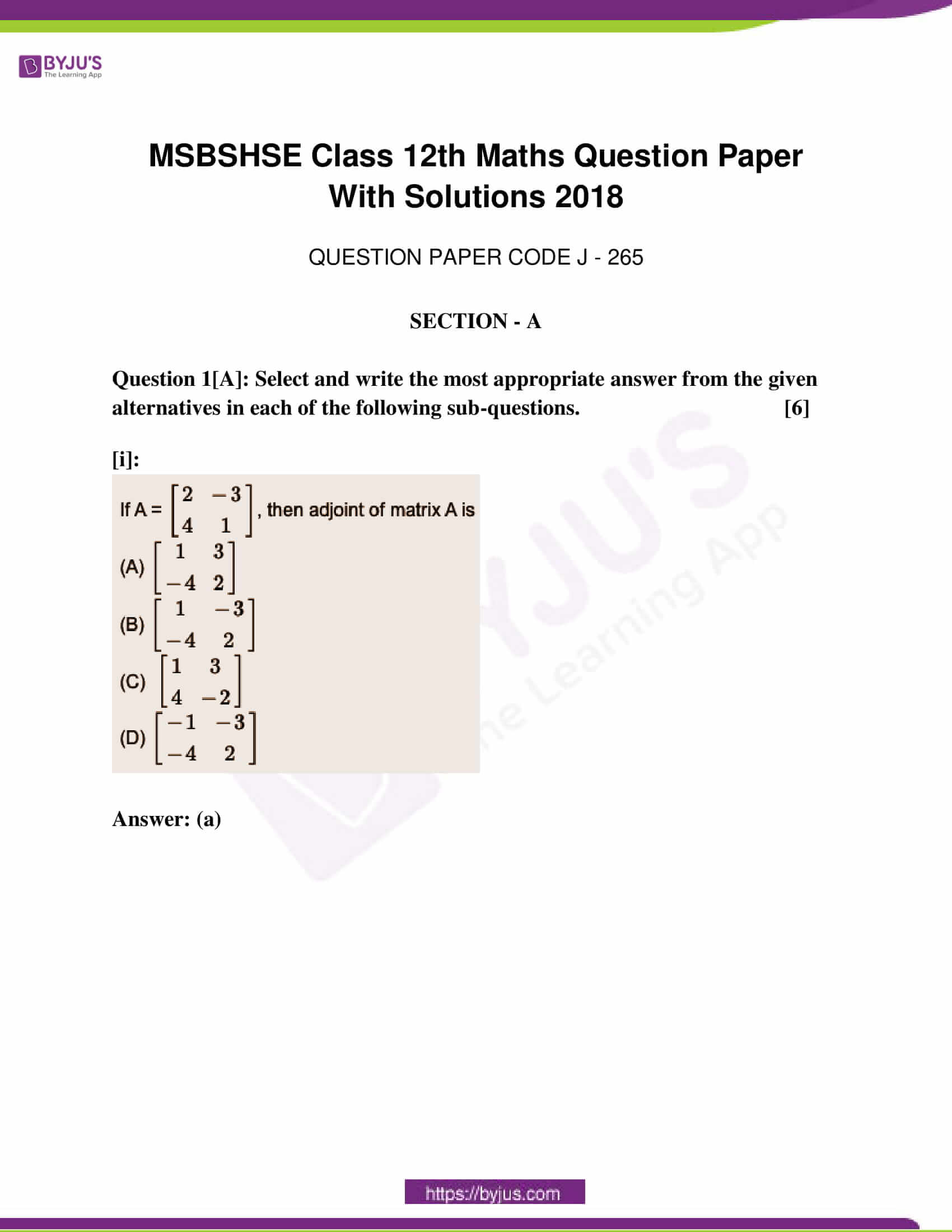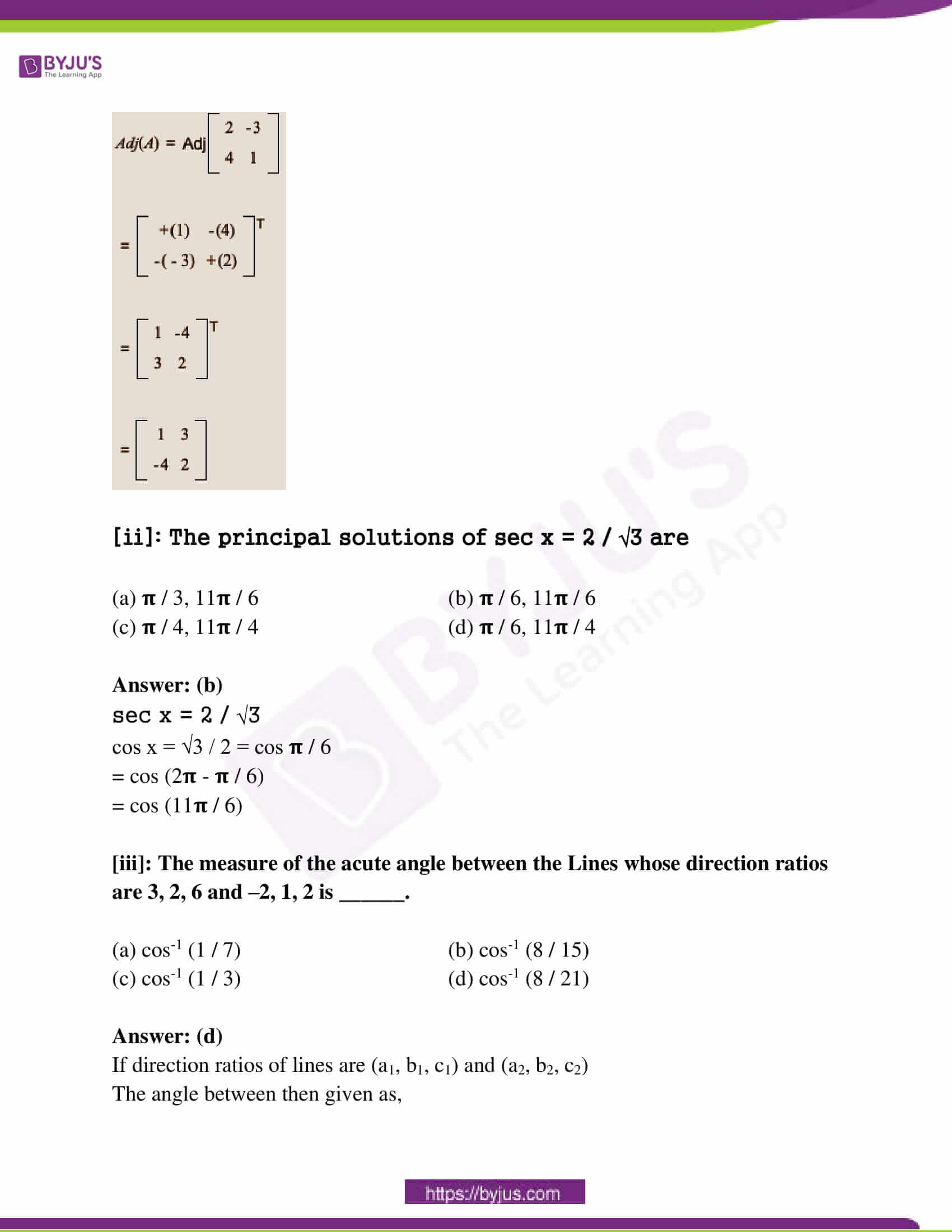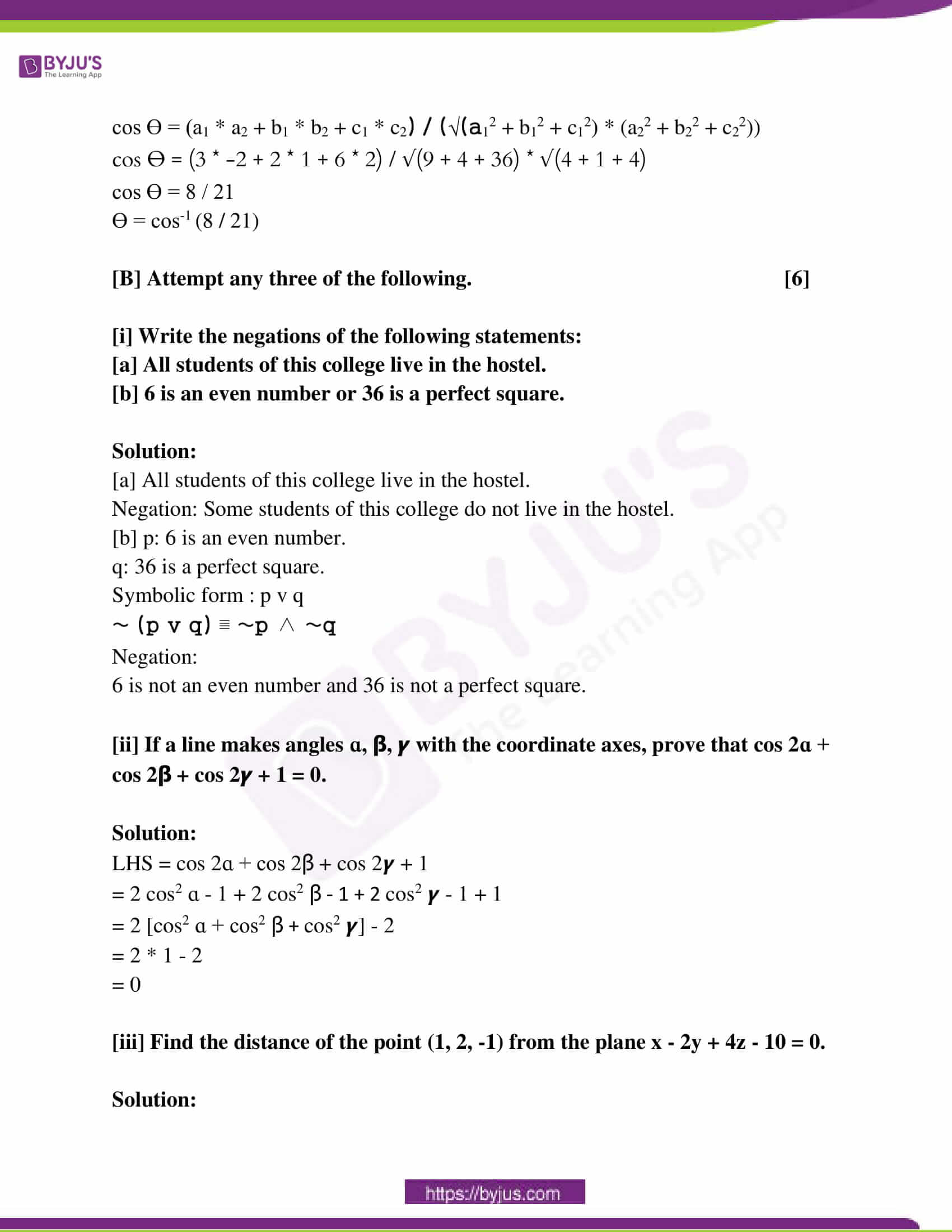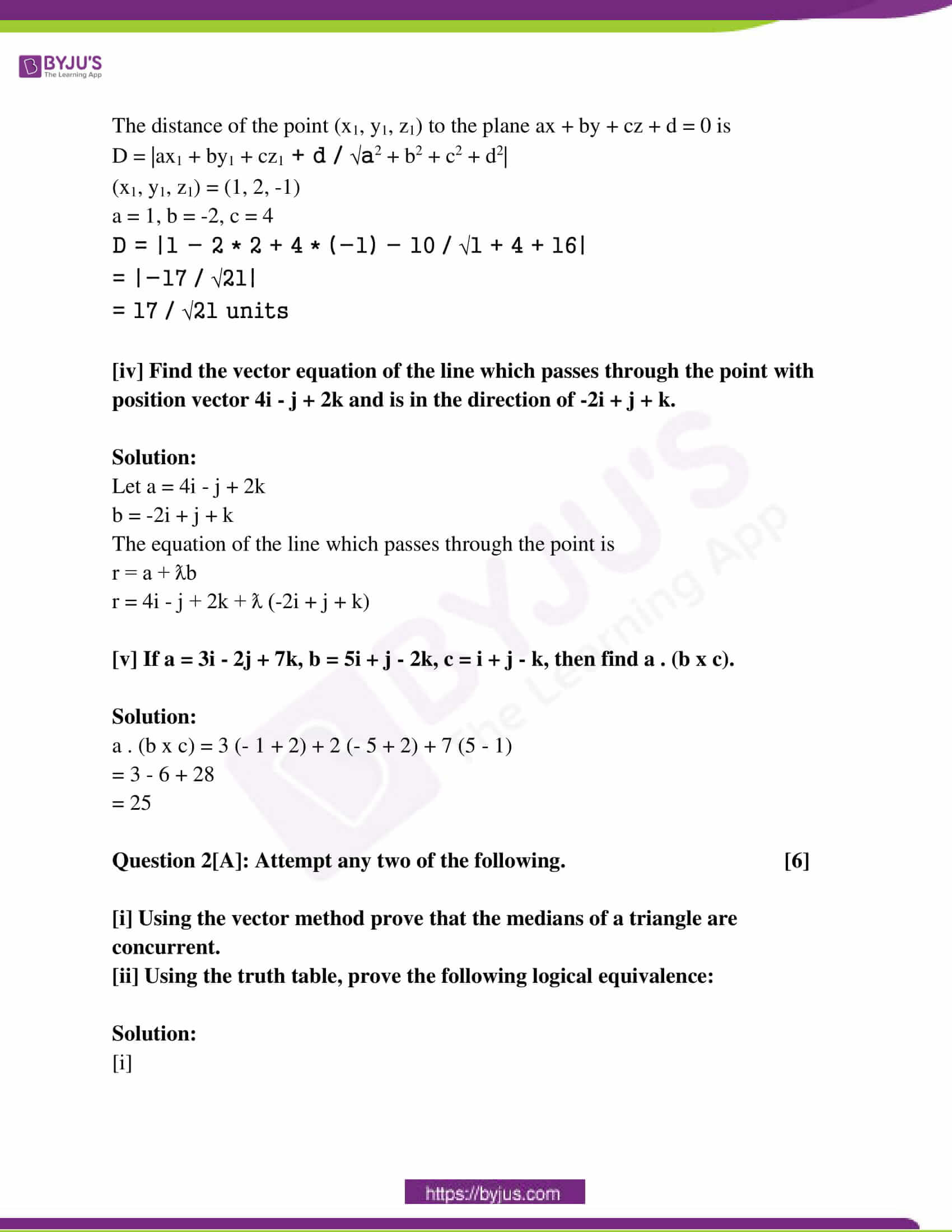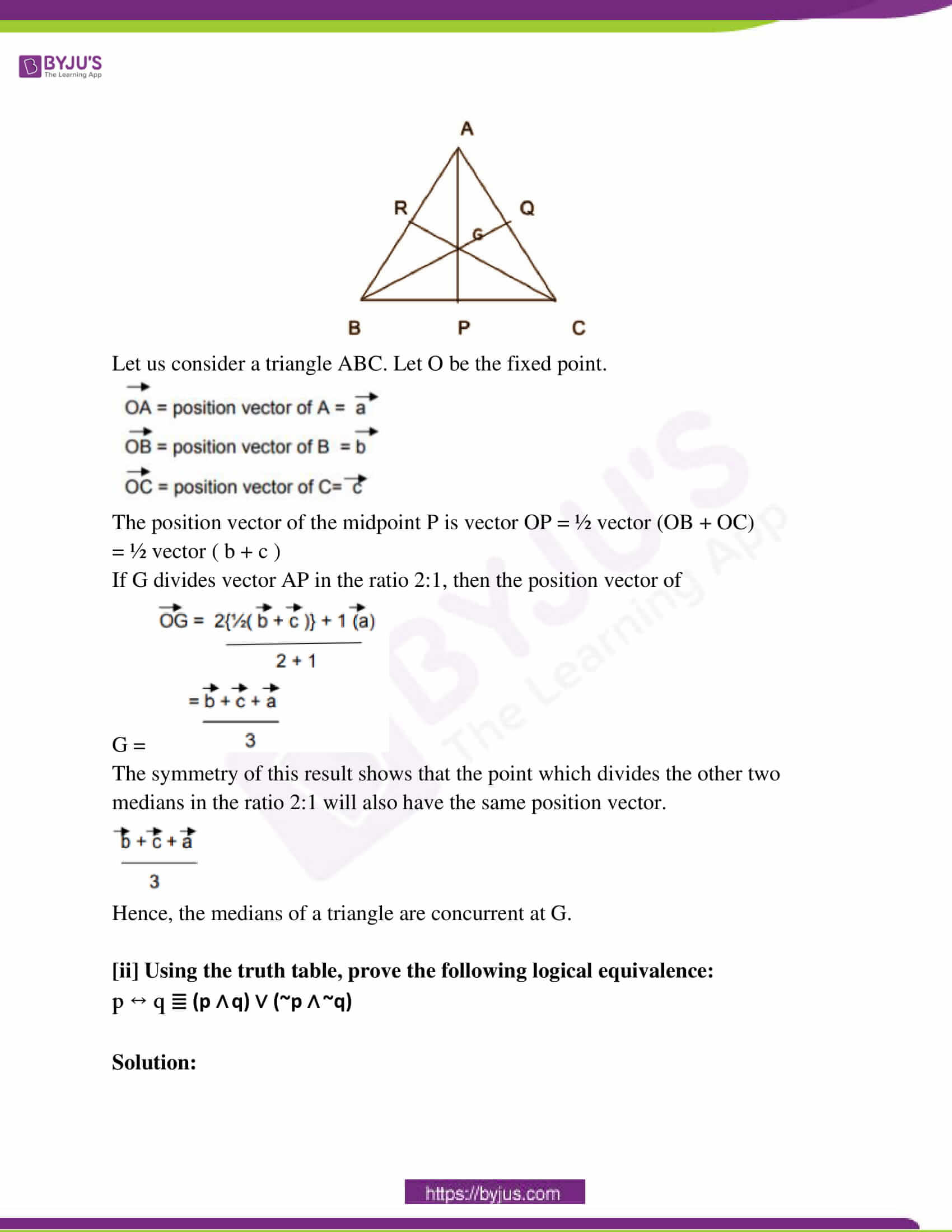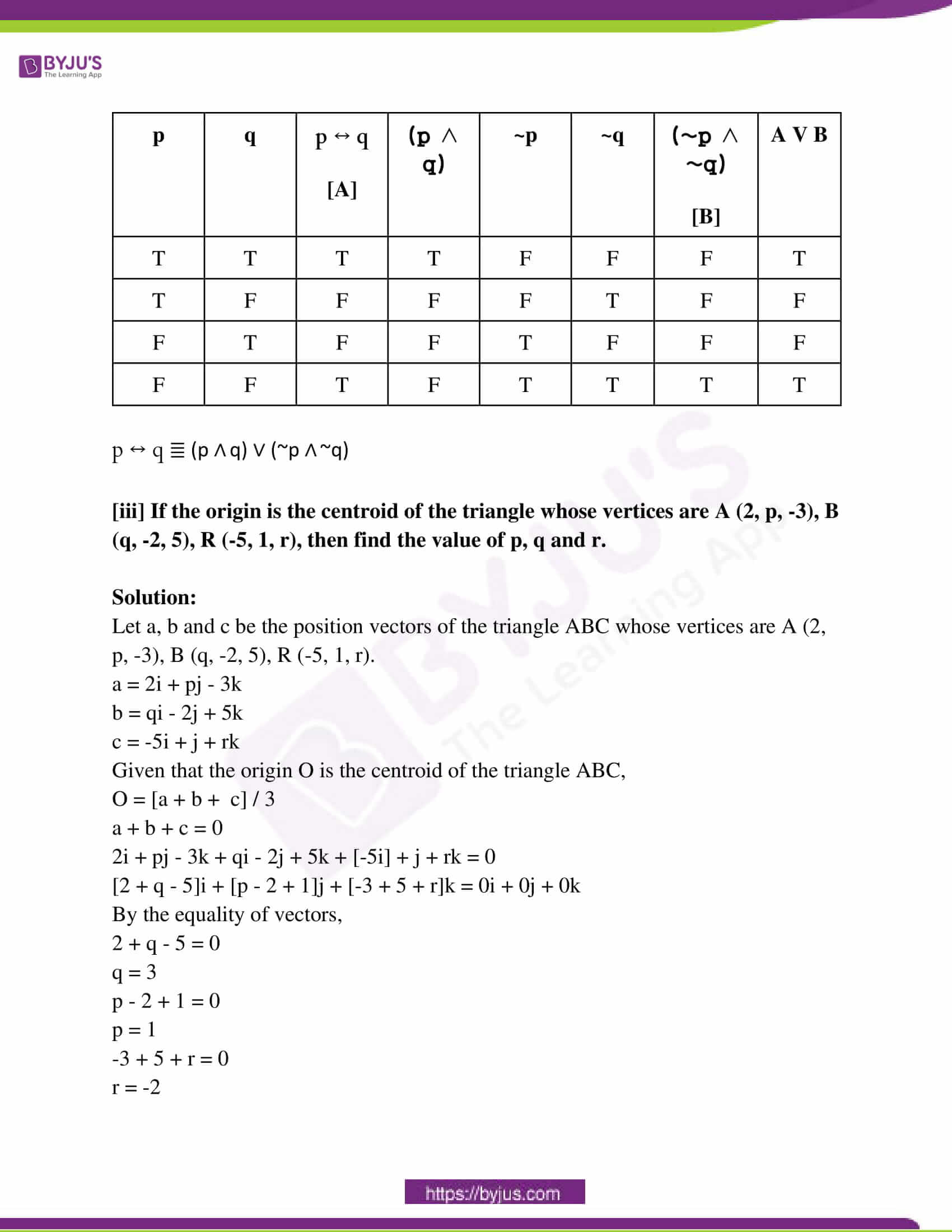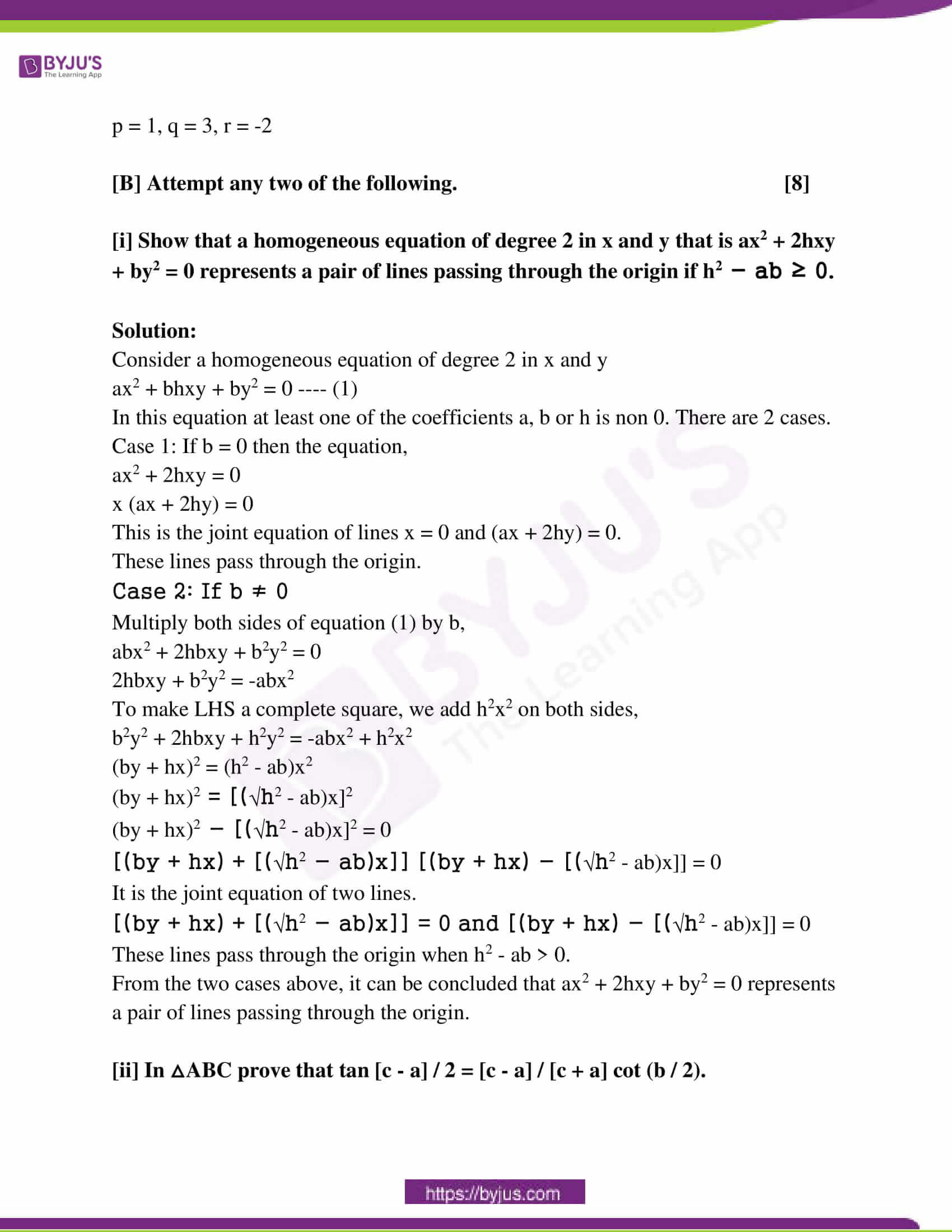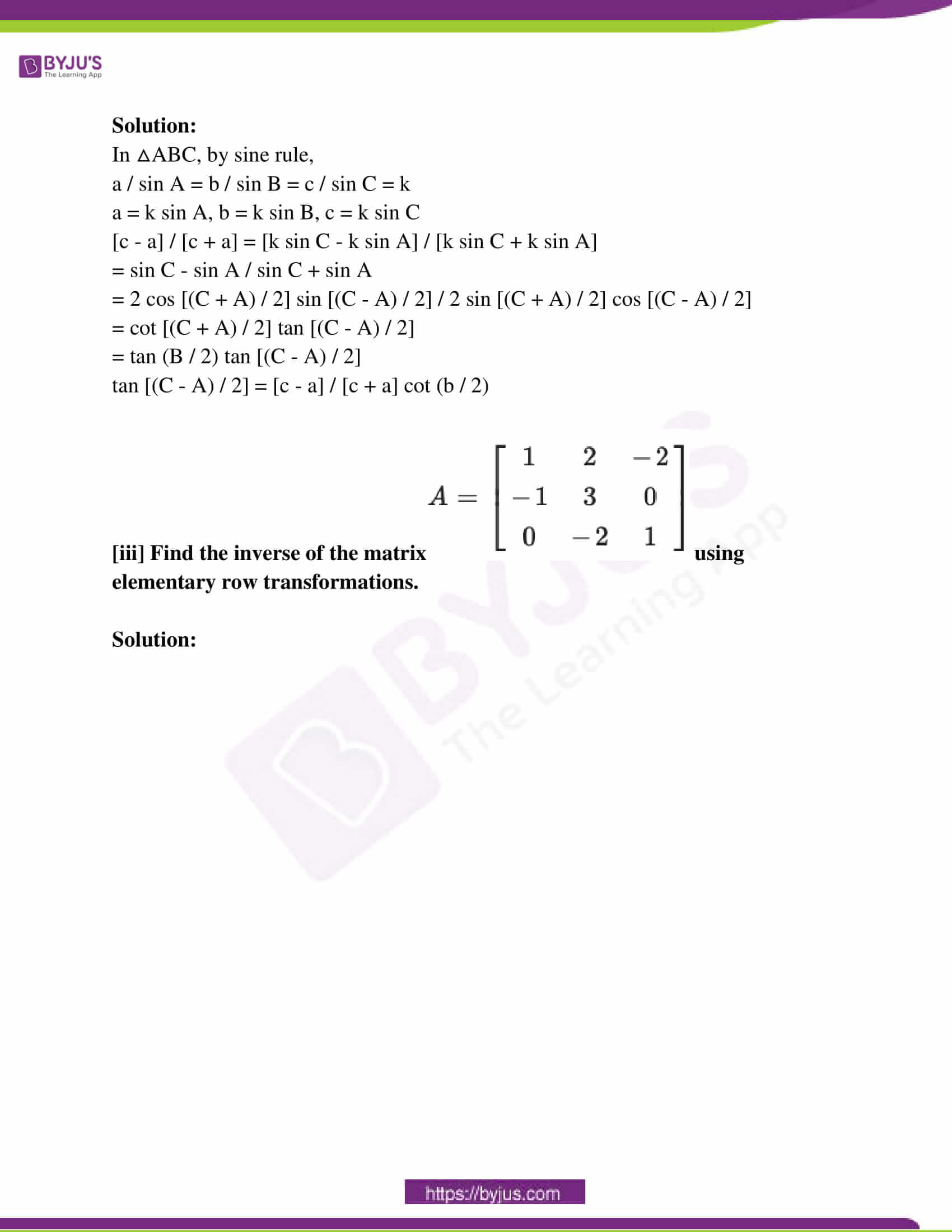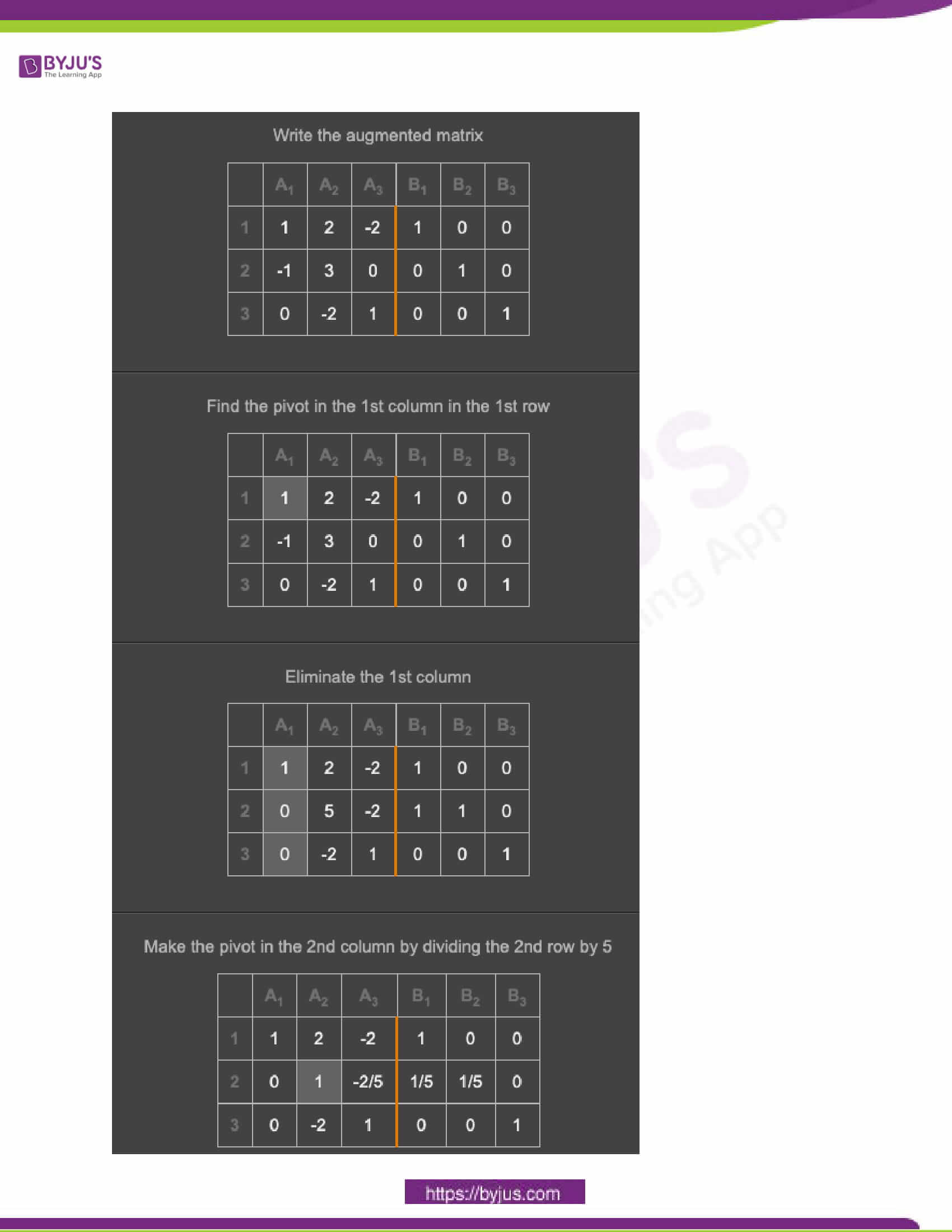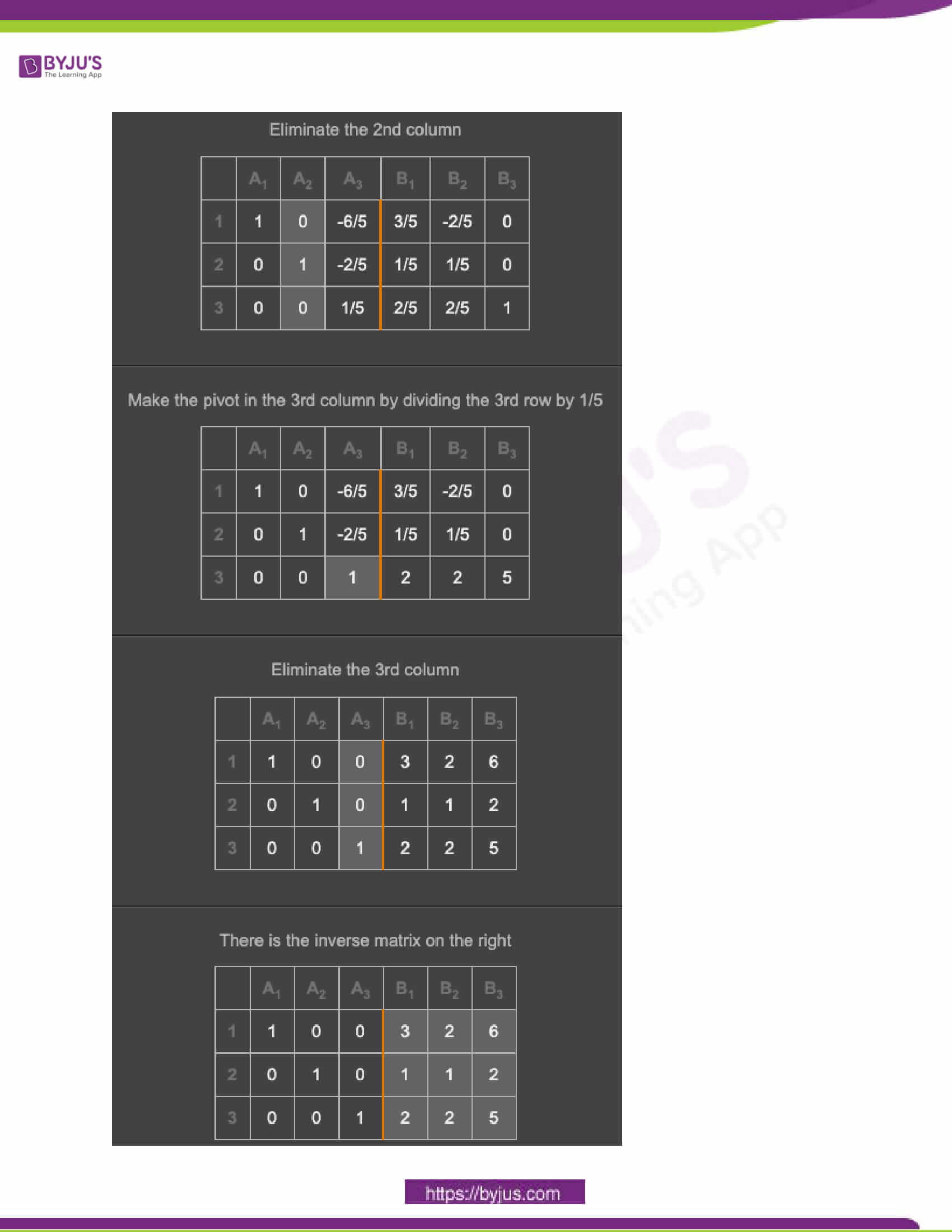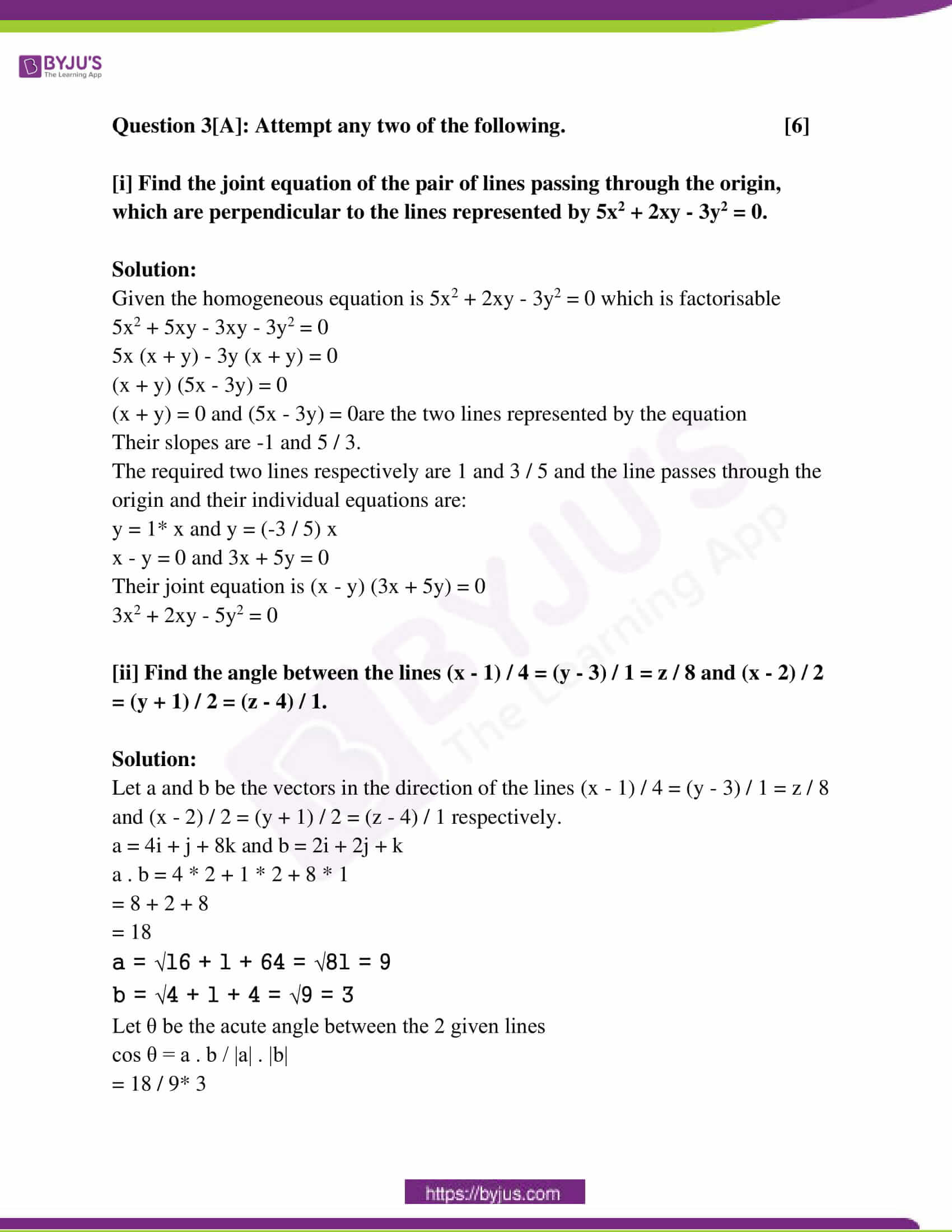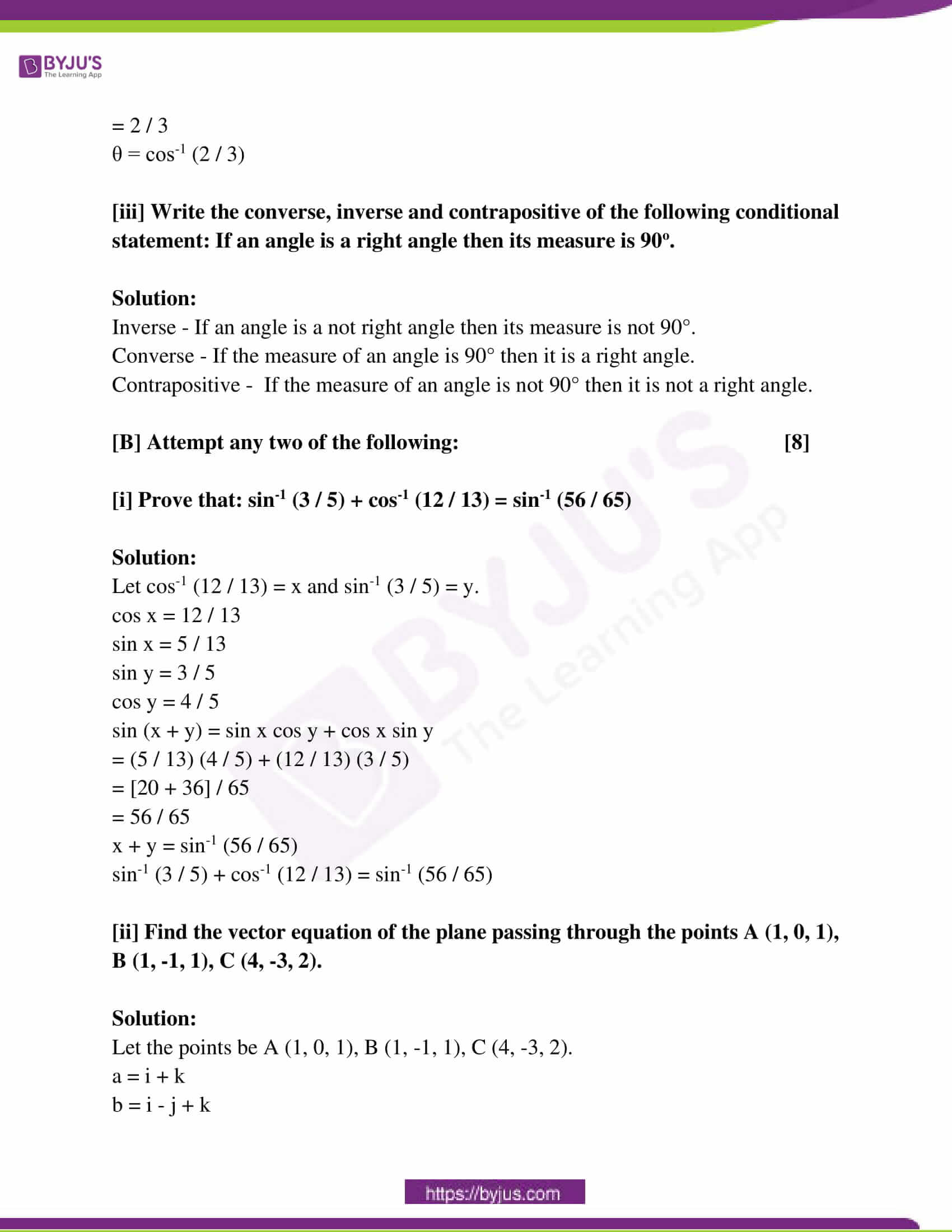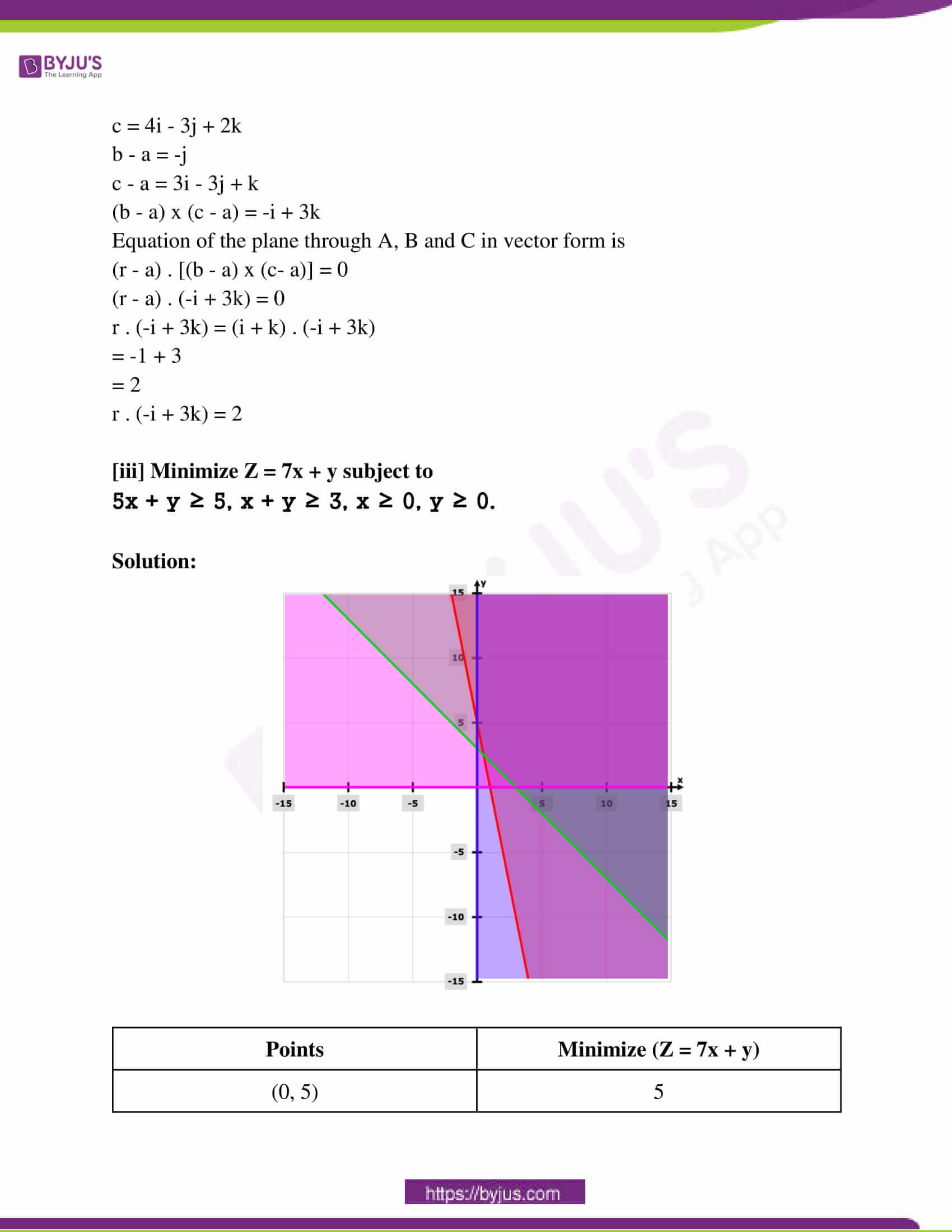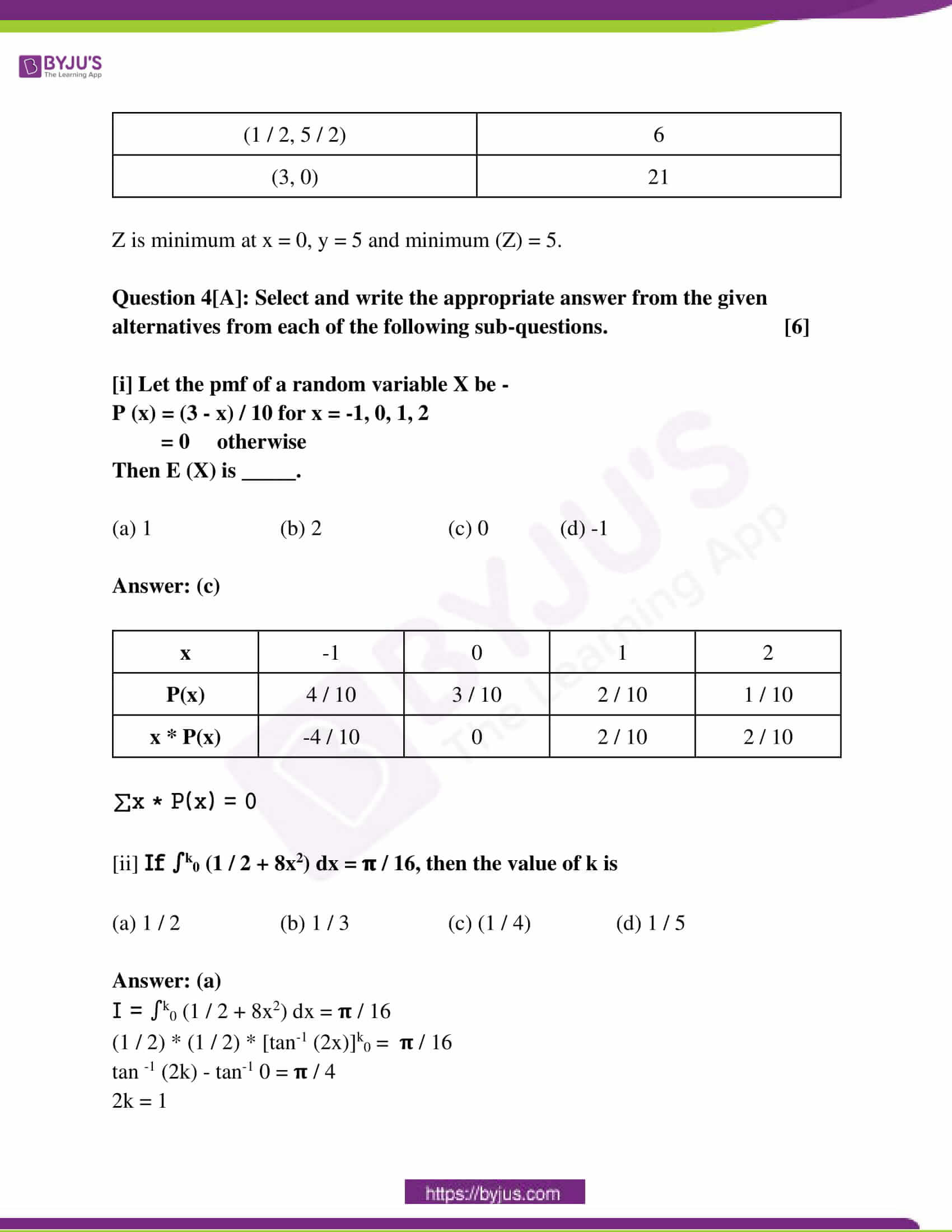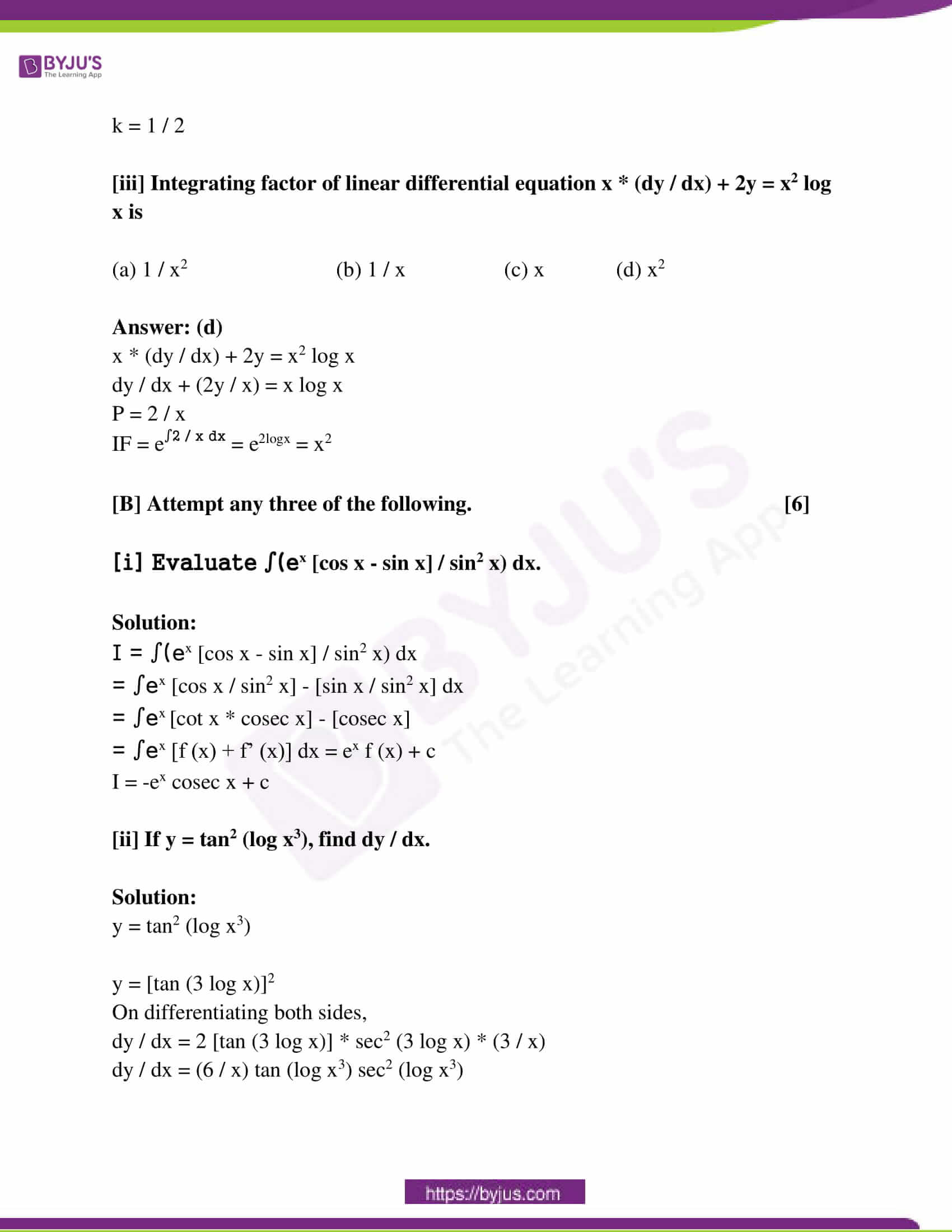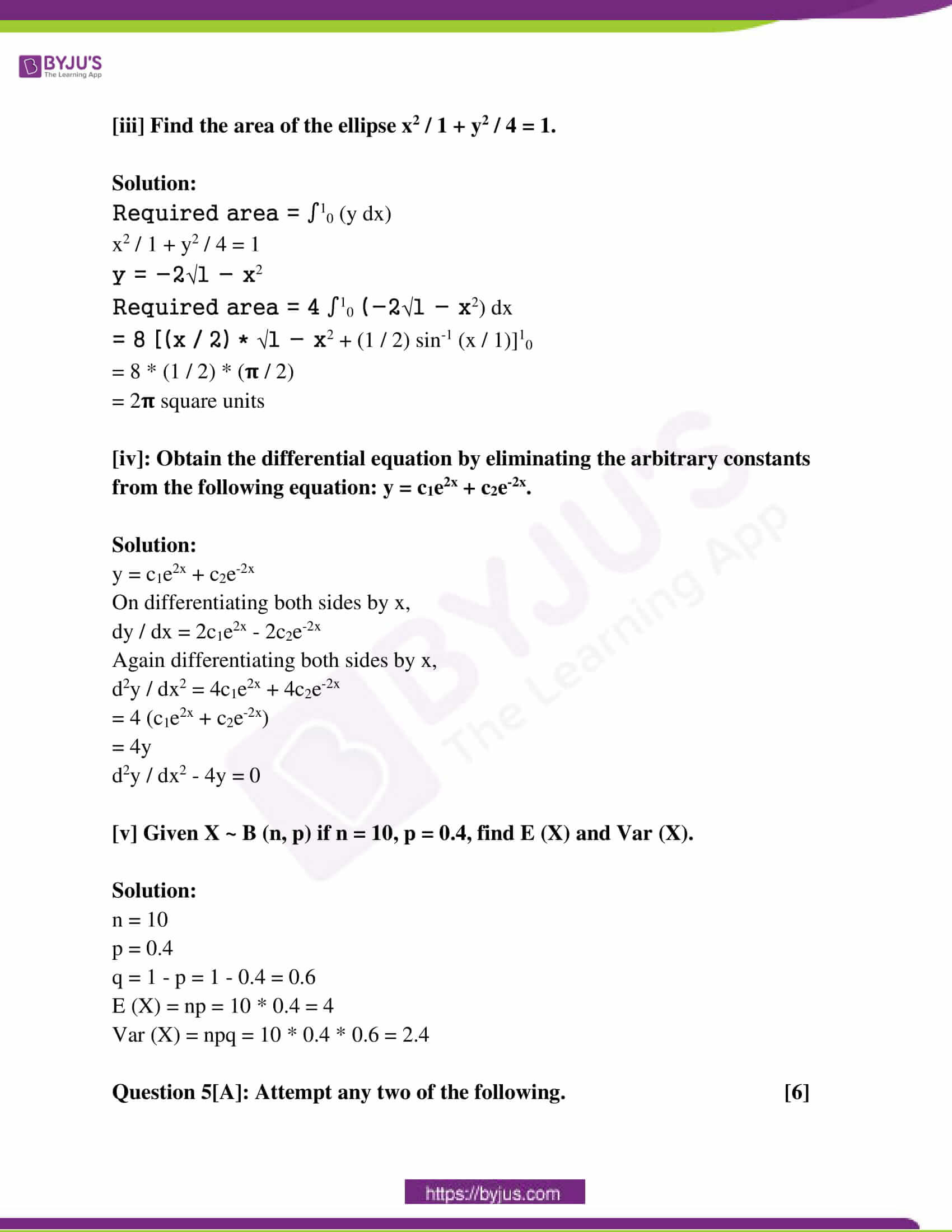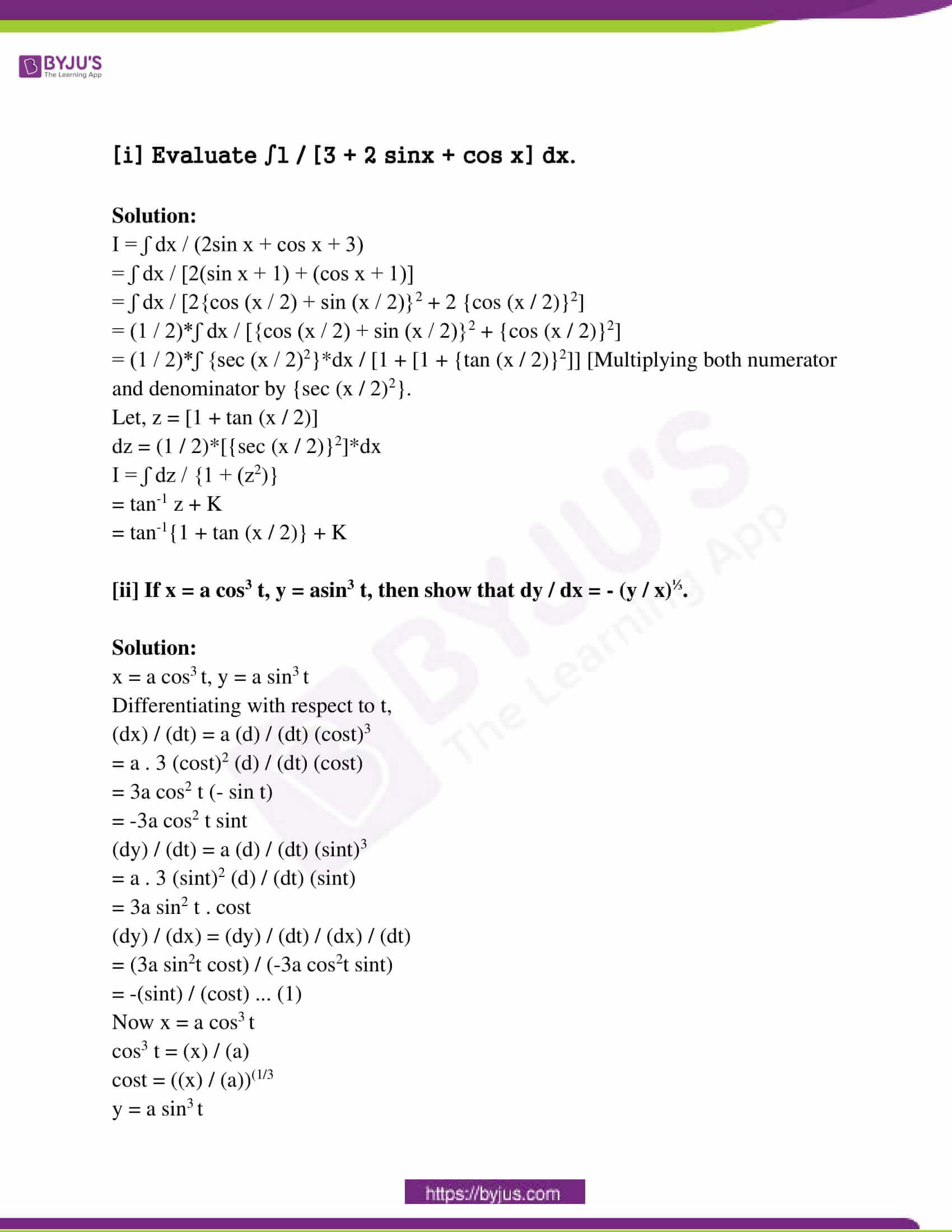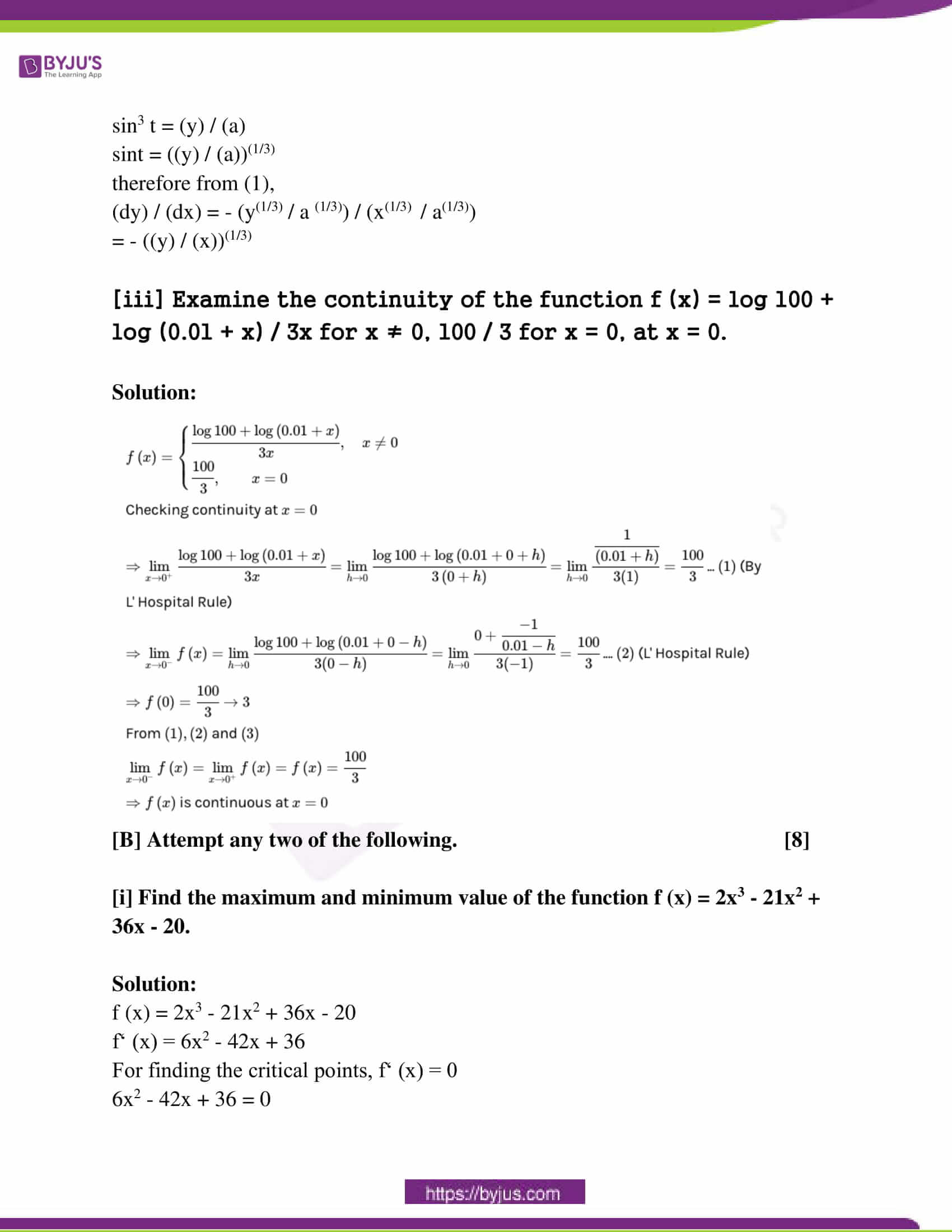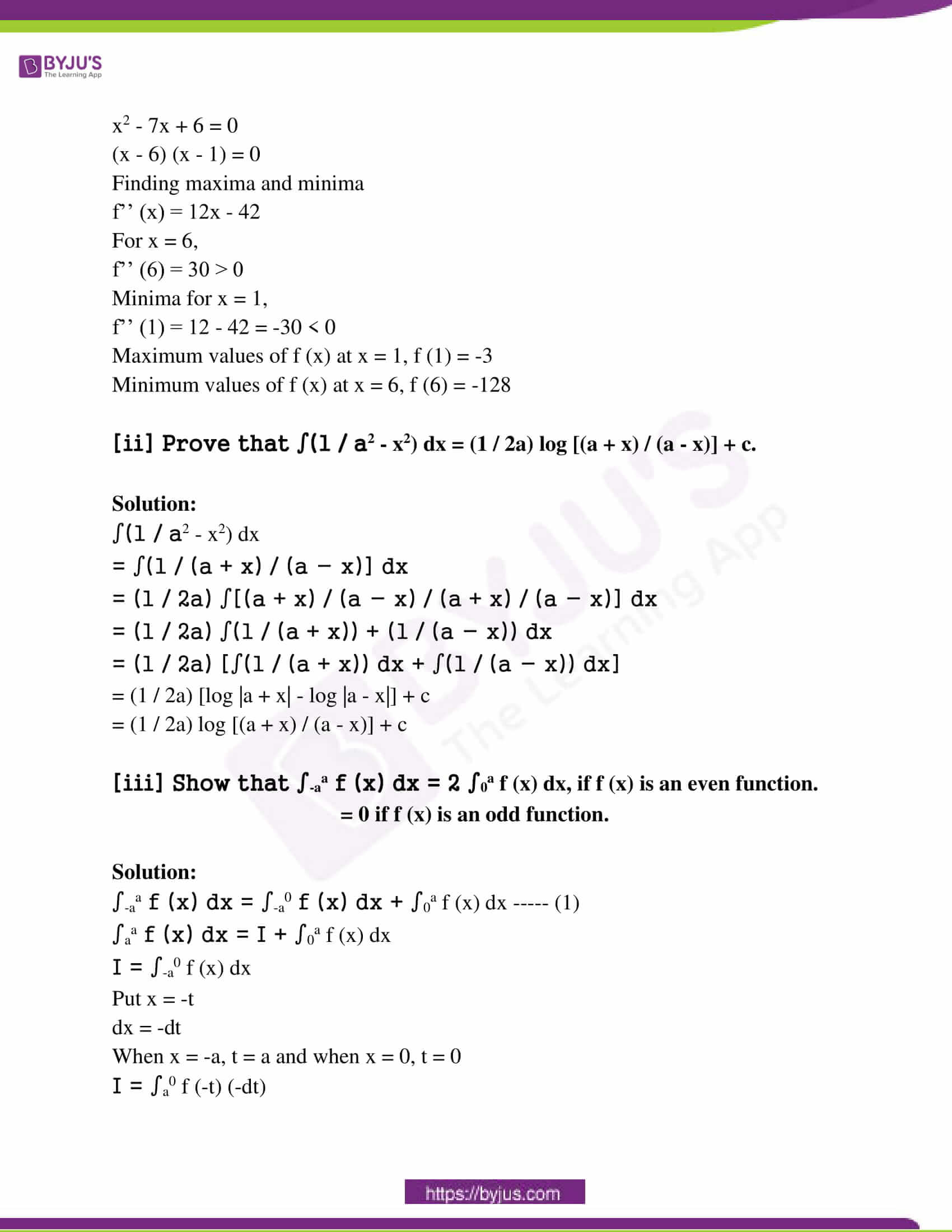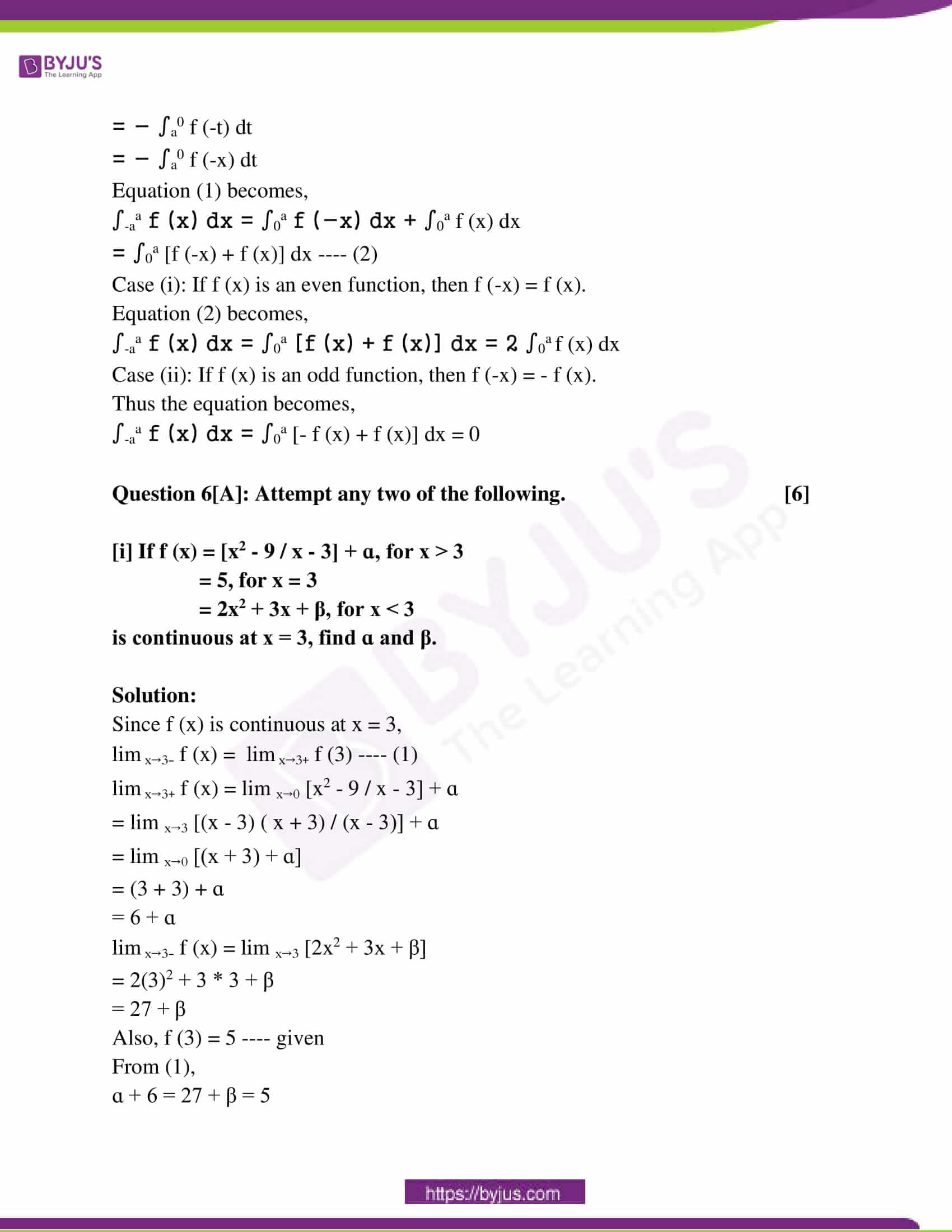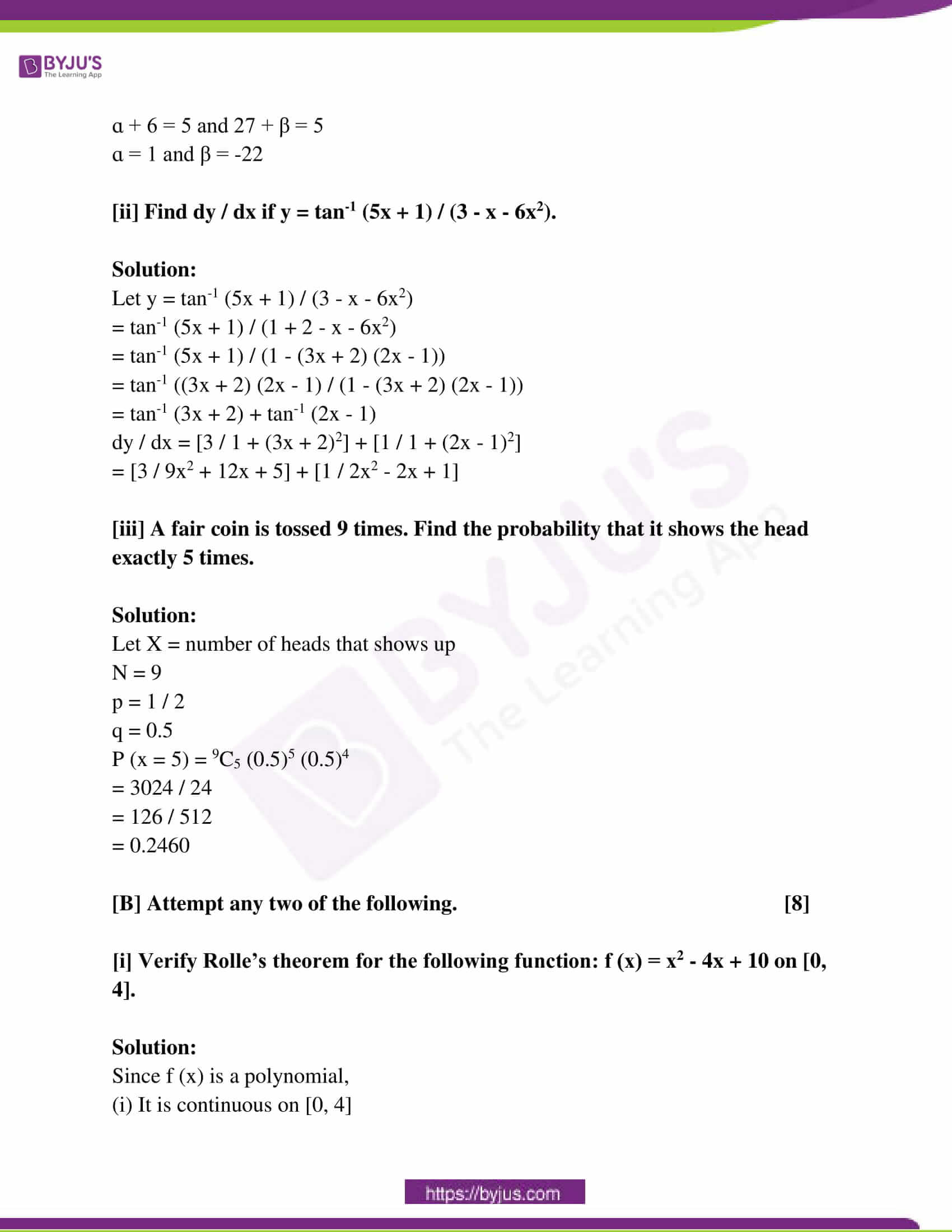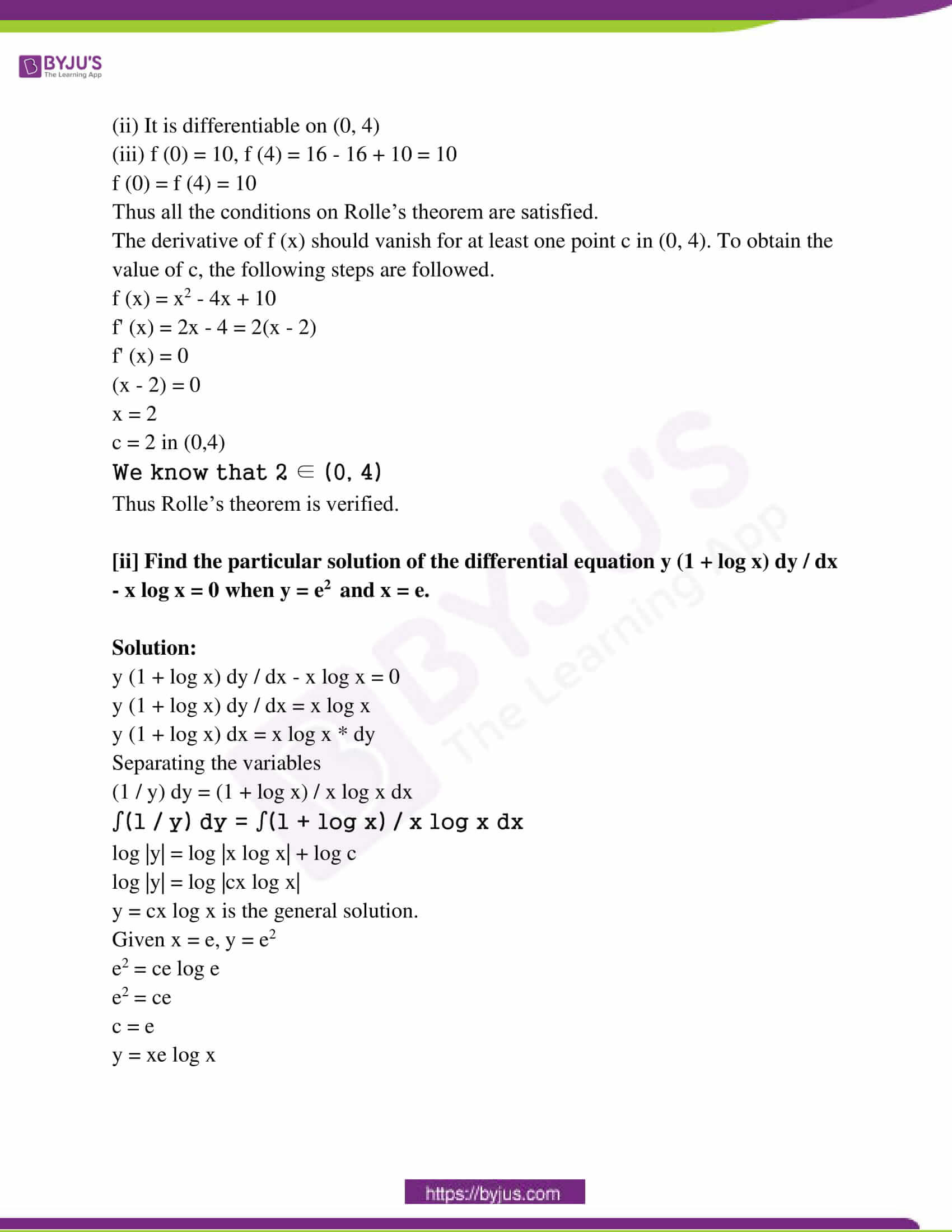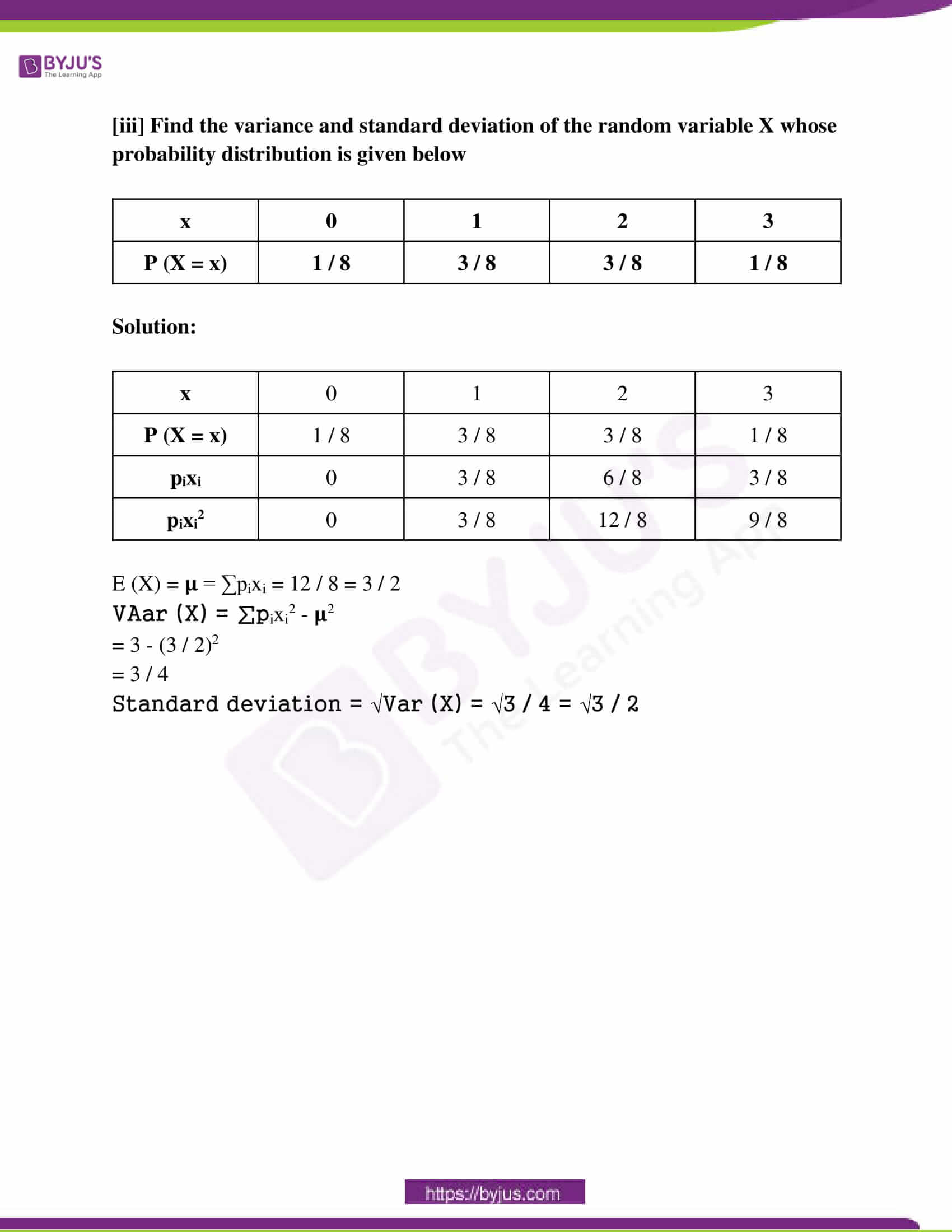SECTION – A

Question 1[A]: Select and write the most appropriate answer from the given alternatives in each of the following sub-questions. 

[i]: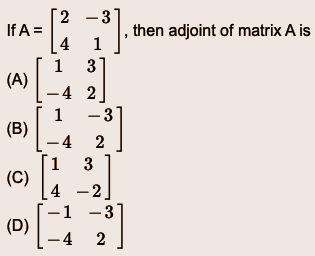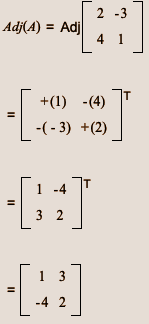[ii]: The principal solutions of sec x = 2 / √3 are

(a) 𝛑 / 3, 11𝛑 / 6 (b) 𝛑 / 6, 11𝛑 / 6

(c) 𝛑 / 4, 11𝛑 / 4 (d) 𝛑 / 6, 11𝛑 / 4

sec x = 2 / √3

cos x = √3 / 2 = cos 𝛑 / 6

= cos (2𝛑 – 𝛑 / 6)

= cos (11𝛑 / 6)

[iii]: The measure of the acute angle between the Lines whose direction ratios are 3, 2, 6 and –2, 1, 2 is ______.

(a) cos-1 (1 / 7) (b) cos-1 (8 / 15)

(c) cos-1 (1 / 3) (d) cos-1 (8 / 21)

If direction ratios of lines are (a1, b1, c1) and (a2, b2, c2)

The angle between then given as,

cos ϴ = (a1 * a2 + b1 * b2 + c1 * c2) / (√(a12 + b12 + c12) * (a22 + b22 + c22))

cos ϴ = (3 * -2 + 2 * 1 + 6 * 2) / √(9 + 4 + 36) * √(4 + 1 + 4)

cos ϴ = 8 / 21

ϴ = cos-1 (8 / 21)

[B] Attempt any three of the following. 

[i] Write the negations of the following statements:

[a] All students of this college live in the hostel.

[b] 6 is an even number or 36 is a perfect square.

Solution:

[a] All students of this college live in the hostel.

Negation: Some students of this college do not live in the hostel.

[b] p: 6 is an even number.

q: 36 is a perfect square.

Symbolic form : p v q

~ (p v q) ≣ ~p ∧ ~q

Negation:

6 is not an even number and 36 is not a perfect square.

[ii] If a line makes angles ɑ, ꞵ, 𝞬 with the coordinate axes, prove that cos 2ɑ + cos 2ꞵ + cos 2𝞬 + 1 = 0.

Solution:

LHS = cos 2ɑ + cos 2ꞵ + cos 2𝞬 + 1

= 2 cos2 ɑ – 1 + 2 cos2 ꞵ – 1 + 2 cos2 𝞬 – 1 + 1

= 2 [cos2 ɑ + cos2 ꞵ + cos2 𝞬] – 2

= 2 * 1 – 2

= 0

[iii] Find the distance of the point (1, 2, -1) from the plane x – 2y + 4z – 10 = 0.

Solution:

The distance of the point (x1, y1, z1) to the plane ax + by + cz + d = 0 is

D = |ax1 + by1 + cz1 + d / √a2 + b2 + c2 + d2|

(x1, y1, z1) = (1, 2, -1)

a = 1, b = -2, c = 4

D = |1 – 2 * 2 + 4 * (-1) – 10 / √1 + 4 + 16|

= |-17 / √21|

= 17 / √21 units

[iv] Find the vector equation of the line which passes through the point with position vector 4i – j + 2k and is in the direction of -2i + j + k.

Solution:

Let a = 4i – j + 2k

b = -2i + j + k

The equation of the line which passes through the point is

r = a + ƛb

r = 4i – j + 2k + ƛ (-2i + j + k)

[v] If a = 3i – 2j + 7k, b = 5i + j – 2k, c = i + j – k, then find a . (b x c).

Solution:

a . (b x c) = 3 (- 1 + 2) + 2 (- 5 + 2) + 7 (5 – 1)

= 3 – 6 + 28

= 25

Question 2[A]: Attempt any two of the following. 

[i] Using the vector method prove that the medians of a triangle are concurrent.

[ii] Using the truth table, prove the following logical equivalence:

Solution:

[i]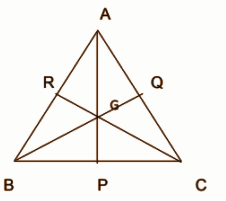Let us consider a triangle ABC. Let O be the fixed point.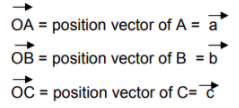The position vector of the midpoint P is vector OP = ½ vector (OB + OC)

= ½ vector ( b + c )

If G divides vector AP in the ratio 2:1, then the position vector of

G =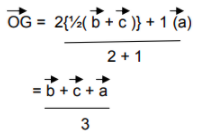The symmetry of this result shows that the point which divides the other two medians in the ratio 2:1 will also have the same position vector.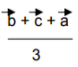Hence, the medians of a triangle are concurrent at G.

[ii] Using the truth table, prove the following logical equivalence:

p ↔ q ≣ (p ∧ q) ∨ (~p ∧ ~q)

Solution:

 p q p ↔ q [A] (p ∧ q) ~p ~q (~p ∧ ~q) [B] A V B T T T T F F F T T F F F F T F F F T F F T F F F F F T F T T T T

p ↔ q ≣ (p ∧ q) ∨ (~p ∧ ~q)

[iii] If the origin is the centroid of the triangle whose vertices are A (2, p, -3), B (q, -2, 5), R (-5, 1, r), then find the value of p, q and r.

Solution:

Let a, b and c be the position vectors of the triangle ABC whose vertices are A (2, p, -3), B (q, -2, 5), R (-5, 1, r).

a = 2i + pj – 3k

b = qi – 2j + 5k

c = -5i + j + rk

Given that the origin O is the centroid of the triangle ABC,

O = [a + b + c] / 3

a + b + c = 0

2i + pj – 3k + qi – 2j + 5k + [-5i] + j + rk = 0

[2 + q – 5]i + [p – 2 + 1]j + [-3 + 5 + r]k = 0i + 0j + 0k

By the equality of vectors,

2 + q – 5 = 0

q = 3

p – 2 + 1 = 0

p = 1

-3 + 5 + r = 0

r = -2

p = 1, q = 3, r = -2

[B] Attempt any two of the following. 

[i] Show that a homogeneous equation of degree 2 in x and y that is ax2 + 2hxy + by2 = 0 represents a pair of lines passing through the origin if h2 – ab ≥ 0.

Solution:

Consider a homogeneous equation of degree 2 in x and y

ax2 + bhxy + by2 = 0 —- (1)

In this equation at least one of the coefficients a, b or h is non 0. There are 2 cases.

Case 1: If b = 0 then the equation,

ax2 + 2hxy = 0

x (ax + 2hy) = 0

This is the joint equation of lines x = 0 and (ax + 2hy) = 0.

These lines pass through the origin.

Case 2: If b ≠ 0

Multiply both sides of equation (1) by b,

abx2 + 2hbxy + b2y2 = 0

2hbxy + b2y2 = -abx2

To make LHS a complete square, we add h2x2 on both sides,

b2y2 + 2hbxy + h2y2 = -abx2 + h2x2

(by + hx)2 = (h2 – ab)x2

(by + hx)2 = [(√h2 – ab)x]2

(by + hx)2 – [(√h2 – ab)x]2 = 0

[(by + hx) + [(√h2 – ab)x]] [(by + hx) – [(√h2 – ab)x]] = 0

It is the joint equation of two lines.

[(by + hx) + [(√h2 – ab)x]] = 0 and [(by + hx) – [(√h2 – ab)x]] = 0

These lines pass through the origin when h2 – ab > 0.

From the two cases above, it can be concluded that ax2 + 2hxy + by2 = 0 represents a pair of lines passing through the origin.

[ii] In △ABC prove that tan [c – a] / 2 = [c – a] / [c + a] cot (b / 2).

Solution:

In △ABC, by sine rule,

a / sin A = b / sin B = c / sin C = k

a = k sin A, b = k sin B, c = k sin C

[c – a] / [c + a] = [k sin C – k sin A] / [k sin C + k sin A]

= sin C – sin A / sin C + sin A

= 2 cos [(C + A) / 2] sin [(C – A) / 2] / 2 sin [(C + A) / 2] cos [(C – A) / 2]

= cot [(C + A) / 2] tan [(C – A) / 2]

= tan (B / 2) tan [(C – A) / 2]

tan [(C – A) / 2] = [c – a] / [c + a] cot (b / 2)

[iii] Find the inverse of the matrix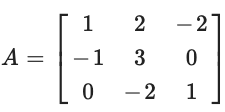using elementary row transformations.

Solution: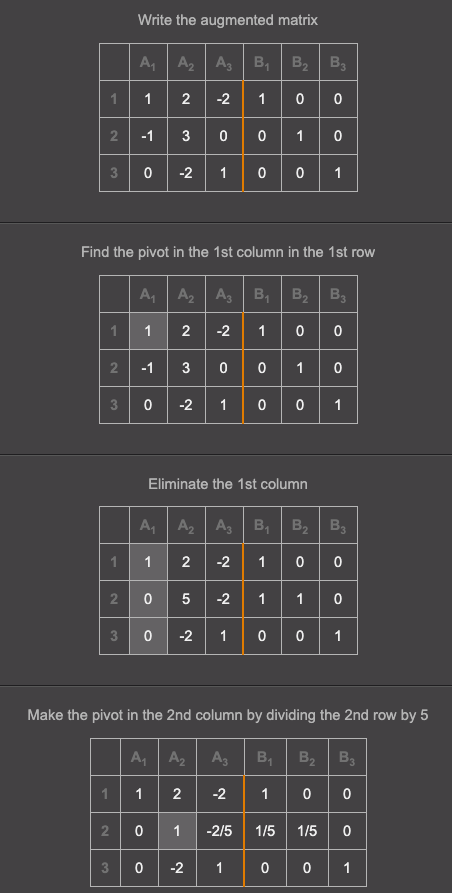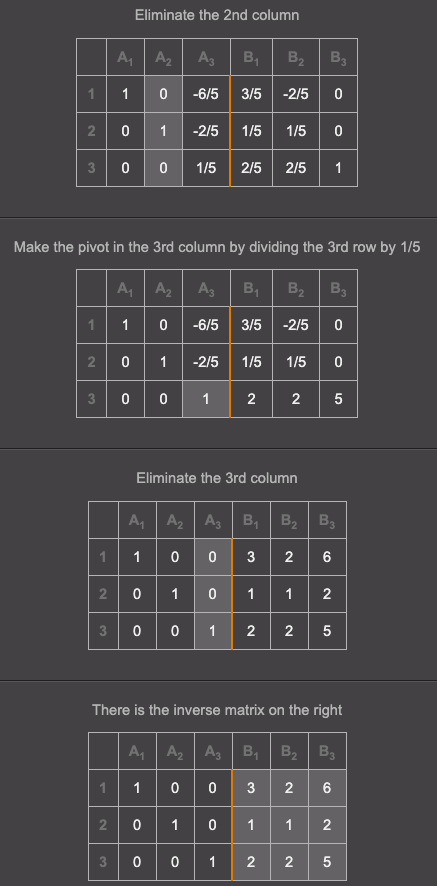Question 3[A]: Attempt any two of the following. 

[i] Find the joint equation of the pair of lines passing through the origin, which are perpendicular to the lines represented by 5x2 + 2xy – 3y2 = 0.

Solution:

Given the homogeneous equation is 5x2 + 2xy – 3y2 = 0 which is factorisable

5x2 + 5xy – 3xy – 3y2 = 0

5x (x + y) – 3y (x + y) = 0

(x + y) (5x – 3y) = 0

(x + y) = 0 and (5x – 3y) = 0are the two lines represented by the equation

Their slopes are -1 and 5 / 3.

The required two lines respectively are 1 and 3 / 5 and the line passes through the origin and their individual equations are:

y = 1* x and y = (-3 / 5) x

x – y = 0 and 3x + 5y = 0

Their joint equation is (x – y) (3x + 5y) = 0

3x2 + 2xy – 5y2 = 0

[ii] Find the angle between the lines (x – 1) / 4 = (y – 3) / 1 = z / 8 and (x – 2) / 2 = (y + 1) / 2 = (z – 4) / 1.

Solution:

Let a and b be the vectors in the direction of the lines (x – 1) / 4 = (y – 3) / 1 = z / 8 and (x – 2) / 2 = (y + 1) / 2 = (z – 4) / 1, respectively.

a = 4i + j + 8k and b = 2i + 2j + k

a . b = 4 * 2 + 1 * 2 + 8 * 1

= 8 + 2 + 8

= 18

a = √16 + 1 + 64 = √81 = 9

b = √4 + 1 + 4 = √9 = 3

Let θ be the acute angle between the 2 given lines

cos θ = a . b / |a| . |b|

= 18 / 9* 3

= 2 / 3

θ = cos-1 (2 / 3)

[iii] Write the converse, inverse and contrapositive of the following conditional statement: If an angle is a right angle then its measure is 90o.

Solution:

Inverse – If an angle is a not right angle then its measure is not 90°.

Converse – If the measure of an angle is 90° then it is a right angle.

Contrapositive – If the measure of an angle is not 90° then it is not a right angle.

[B] Attempt any two of the following: 

[i] Prove that: sin-1 (3 / 5) + cos-1 (12 / 13) = sin-1 (56 / 65)

Solution:

Let cos-1 (12 / 13) = x and sin-1 (3 / 5) = y.

cos x = 12 / 13

sin x = 5 / 13

sin y = 3 / 5

cos y = 4 / 5

sin (x + y) = sin x cos y + cos x sin y

= (5 / 13) (4 / 5) + (12 / 13) (3 / 5)

= [20 + 36] / 65

= 56 / 65

x + y = sin-1 (56 / 65)

sin-1 (3 / 5) + cos-1 (12 / 13) = sin-1 (56 / 65)

[ii] Find the vector equation of the plane passing through the points A (1, 0, 1), B (1, -1, 1), C (4, -3, 2).

Solution:

Let the points be A (1, 0, 1), B (1, -1, 1), C (4, -3, 2).

a = i + k

b = i – j + k

c = 4i – 3j + 2k

b – a = -j

c – a = 3i – 3j + k

(b – a) x (c – a) = -i + 3k

Equation of the plane through A, B and C in vector form is

(r – a) . [(b – a) x (c- a)] = 0

(r – a) . (-i + 3k) = 0

r . (-i + 3k) = (i + k) . (-i + 3k)

= -1 + 3

= 2

r . (-i + 3k) = 2

[iii] Minimize Z = 7x + y subject to

5x + y ≥ 5, x + y ≥ 3, x ≥ 0, y ≥ 0.

Solution: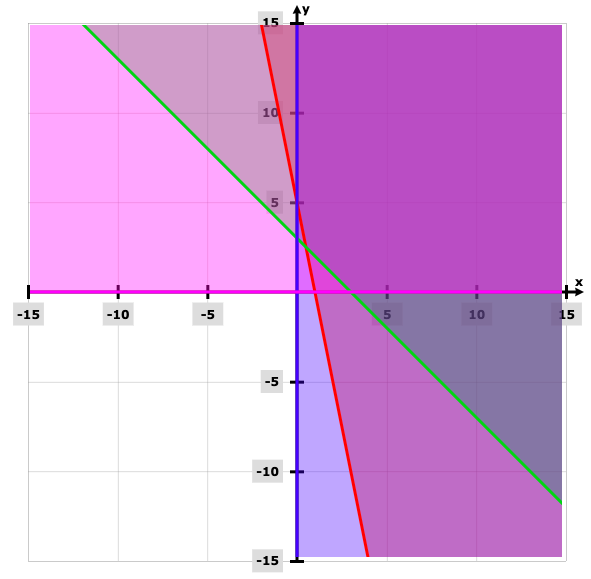Points Minimize (Z = 7x + y) (0, 5) 5 (1 / 2, 5 / 2) 6 (3, 0) 21

Z is minimum at x = 0, y = 5 and minimum (Z) = 5.

Question 4[A]: Select and write the appropriate answer from the given alternatives from each of the following sub-questions. 

[i] Let the pmf of a random variable X be –

P (x) = (3 – x) / 10 for x = -1, 0, 1, 2

= 0 otherwise

Then E (X) is _____.

(a) 1 (b) 2 (c) 0 (d) -1

 x -1 0 1 2 P(x) 4 / 10 3 / 10 2 / 10 1 / 10 x * P(x) -4 / 10 0 2 / 10 2 / 10

∑x * P(x) = 0

[ii] If ∫k0 (1 / 2 + 8x2) dx = 𝛑 / 16, then the value of k is

(a) 1 / 2 (b) 1 / 3 (c) (1 / 4) (d) 1 / 5

I = ∫k0 (1 / 2 + 8x2) dx = 𝛑 / 16

(1 / 2) * (1 / 2) * [tan-1 (2x)]k0 = 𝛑 / 16

tan -1 (2k) – tan-1 0 = 𝛑 / 4

2k = 1

k = 1 / 2

[iii] Integrating factor of linear differential equation x * (dy / dx) + 2y = x2 log x is

(a) 1 / x2 (b) 1 / x (c) x (d) x2

x * (dy / dx) + 2y = x2 log x

dy / dx + (2y / x) = x log x

P = 2 / x

IF = e∫2 / x dx = e2logx = x2

[B] Attempt any three of the following. 

[i] Evaluate ∫(ex [cos x – sin x] / sin2 x) dx.

Solution:

I = ∫(ex [cos x – sin x] / sin2 x) dx

= ∫ex [cos x / sin2 x] – [sin x / sin2 x] dx

= ∫ex [cot x * cosec x] – [cosec x]

= ∫ex [f (x) + f’ (x)] dx = ex f (x) + c

I = -ex cosec x + c

[ii] If y = tan2 (log x3), find dy / dx.

Solution:

y = tan2 (log x3)

y = [tan (3 log x)]2

On differentiating both sides,

dy / dx = 2 [tan (3 log x)] * sec2 (3 log x) * (3 / x)

dy / dx = (6 / x) tan (log x3) sec2 (log x3)

[iii] Find the area of the ellipse x2 / 1 + y2 / 4 = 1.

Solution:

Required area = ∫10 (y dx)

x2 / 1 + y2 / 4 = 1

y = -2√1 – x2

Required area = 4 ∫10 (-2√1 – x2) dx

= 8 [(x / 2) * √1 – x2 + (1 / 2) sin-1 (x / 1)]10

= 8 * (1 / 2) * (𝛑 / 2)

= 2𝛑 square units

[iv]: Obtain the differential equation by eliminating the arbitrary constants from the following equation: y = c1e2x + c2e-2x.

Solution:

y = c1e2x + c2e-2x

On differentiating both sides by x,

dy / dx = 2c1e2x – 2c2e-2x

Again differentiating both sides by x,

d2y / dx2 = 4c1e2x + 4c2e-2x

= 4 (c1e2x + c2e-2x)

= 4y

d2y / dx2 – 4y = 0

[v] Given X ~ B (n, p) if n = 10, p = 0.4, find E (X) and Var (X).

Solution:

n = 10

p = 0.4

q = 1 – p = 1 – 0.4 = 0.6

E (X) = np = 10 * 0.4 = 4

Var (X) = npq = 10 * 0.4 * 0.6 = 2.4

Question 5[A]: Attempt any two of the following. 

[i] Evaluate ∫1 / [3 + 2 sinx + cos x] dx.

Solution:

I = ʃ dx / (2sin x + cos x + 3)

= ʃ dx / [2(sin x + 1) + (cos x + 1)]

= ʃ dx / [2{cos (x / 2) + sin (x / 2)}2 + 2 {cos (x / 2)}2]

= (1 / 2)*ʃ dx / [{cos (x / 2) + sin (x / 2)}2 + {cos (x / 2)}2]

= (1 / 2)*ʃ {sec (x / 2)2}*dx / [1 + [1 + {tan (x / 2)}2]] [Multiplying both numerator and denominator by {sec (x / 2)2}.

Let, z = [1 + tan (x / 2)]

dz = (1 / 2)*[{sec (x / 2)}2]*dx

I = ʃ dz / {1 + (z2)}

= tan-1 z + K

= tan-1{1 + tan (x / 2)} + K

[ii] If x = a cos3 t, y = asin3 t, then show that dy / dx = – (y / x).

Solution:

x = a cos3 t, y = a sin3 t

Differentiating with respect to t,

(dx) / (dt) = a (d) / (dt) (cost)3

= a . 3 (cost)2 (d) / (dt) (cost)

= 3a cos2 t (- sin t)

= -3a cos2 t sint

(dy) / (dt) = a (d) / (dt) (sint)3

= a . 3 (sint)2 (d) / (dt) (sint)

= 3a sin2 t . cost

(dy) / (dx) = (dy) / (dt) / (dx) / (dt)

= (3a sin2t cost) / (-3a cos2t sint)

= -(sint) / (cost) … (1)

Now x = a cos3 t

cos3 t = (x) / (a)

cost = ((x) / (a))(1/3

y = a sin3 t

sin3 t = (y) / (a)

sint = ((y) / (a))(1/3)

therefore from (1),

(dy) / (dx) = – (y(1/3) / a (1/3)) / (x(1/3) / a(1/3))

= – ((y) / (x))(1/3)

[iii] Examine the continuity of the function f (x) = log 100 + log (0.01 + x) / 3x for x ≠ 0, 100 / 3 for x = 0, at x = 0.

Solution: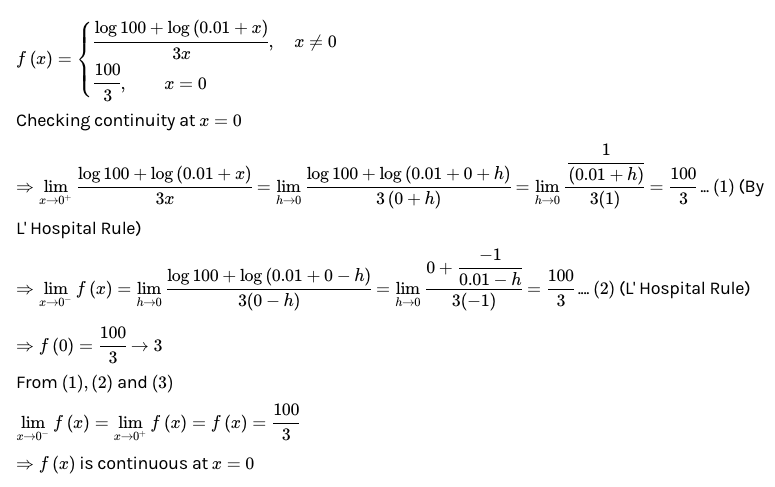[B] Attempt any two of the following. 

[i] Find the maximum and minimum value of the function f (x) = 2x3 – 21x2 + 36x – 20.

Solution:

f (x) = 2x3 – 21x2 + 36x – 20

f‘ (x) = 6x2 – 42x + 36

For finding the critical points, f‘ (x) = 0

6x2 – 42x + 36 = 0

x2 – 7x + 6 = 0

(x – 6) (x – 1) = 0

Finding maxima and minima

f’’ (x) = 12x – 42

For x = 6,

f’’ (6) = 30 > 0

Minima for x = 1,

f’’ (1) = 12 – 42 = -30 < 0

Maximum values of f (x) at x = 1, f (1) = -3

Minimum values of f (x) at x = 6, f (6) = -128

[ii] Prove that ∫(1 / a2 – x2) dx = (1 / 2a) log [(a + x) / (a – x)] + c.

Solution:

∫(1 / a2 – x2) dx

= ∫(1 / (a + x) / (a – x)] dx

= (1 / 2a) ∫[(a + x) / (a – x) / (a + x) / (a – x)] dx

= (1 / 2a) ∫(1 / (a + x)) + (1 / (a – x)) dx

= (1 / 2a) [∫(1 / (a + x)) dx + ∫(1 / (a – x)) dx]

= (1 / 2a) [log |a + x| – log |a – x|] + c

= (1 / 2a) log [(a + x) / (a – x)] + c

[iii] Show that ∫-aa f (x) dx = 2 ∫0a f (x) dx, if f (x) is an even function.

= 0 if f (x) is an odd function.

Solution:

-aa f (x) dx = ∫-a0 f (x) dx + ∫0a f (x) dx —– (1)

aa f (x) dx = I + ∫0a f (x) dx

I = ∫-a0 f (x) dx

Put x = -t

dx = -dt

When x = -a, t = a and when x = 0, t = 0

I = ∫a0 f (-t) (-dt)

= – ∫a0 f (-t) dt

= – ∫a0 f (-x) dt

Equation (1) becomes,

-aa f (x) dx = ∫0a f (-x) dx + ∫0a f (x) dx

= ∫0a [f (-x) + f (x)] dx —- (2)

Case (i): If f (x) is an even function, then f (-x) = f (x).

Equation (2) becomes,

-aa f (x) dx = ∫0a [f (x) + f (x)] dx = 2 ∫0a f (x) dx

Case (ii): If f (x) is an odd function, then f (-x) = – f (x).

Thus the equation becomes,

-aa f (x) dx = ∫0a [- f (x) + f (x)] dx = 0

Question 6[A]: Attempt any two of the following. 

[i] If f (x) = [x2 – 9 / x – 3] + ɑ, for x > 3

= 5, for x = 3

= 2x2 + 3x + β, for x < 3

is continuous at x = 3, find ɑ and β.

Solution:

Since f (x) is continuous at x = 3,

lim x→3- f (x) = lim x→3+ f (3) —- (1)

lim x→3+ f (x) = lim x→0 [x2 – 9 / x – 3] + ɑ

= lim x→3 [(x – 3) ( x + 3) / (x – 3)] + ɑ

= lim x→0 [(x + 3) + ɑ]

= (3 + 3) + ɑ

= 6 + ɑ

lim x→3- f (x) = lim x→3 [2x2 + 3x + β]

= 2(3)2 + 3 * 3 + β

= 27 + β

Also, f (3) = 5 —- given

From (1),

ɑ + 6 = 27 + β = 5

ɑ + 6 = 5 and 27 + β = 5

ɑ = 1 and β = -22

[ii] Find dy / dx if y = tan-1 (5x + 1) / (3 – x – 6x2).

Solution:

Let y = tan-1 (5x + 1) / (3 – x – 6x2)

= tan-1 (5x + 1) / (1 + 2 – x – 6x2)

= tan-1 (5x + 1) / (1 – (3x + 2) (2x – 1))

= tan-1 ((3x + 2) (2x – 1) / (1 – (3x + 2) (2x – 1))

= tan-1 (3x + 2) + tan-1 (2x – 1)

dy / dx = [3 / 1 + (3x + 2)2] + [1 / 1 + (2x – 1)2]

= [3 / 9x2 + 12x + 5] + [1 / 2x2 – 2x + 1]

[iii] A fair coin is tossed 9 times. Find the probability that it shows the head exactly 5 times.

Solution:

Let X = number of heads that shows up

N = 9

p = 1 / 2

q = 0.5

P (x = 5) = 9C5 (0.5)5 (0.5)4

= 3024 / 24

= 126 / 512

= 0.2460

[B] Attempt any two of the following. 

[i] Verify Rolle’s theorem for the following function: f (x) = x2 – 4x + 10 on [0, 4].

Solution:

Since f (x) is a polynomial,

(i) It is continuous on [0, 4]

(ii) It is differentiable on (0, 4)

(iii) f (0) = 10, f (4) = 16 – 16 + 10 = 10

f (0) = f (4) = 10

Thus all the conditions on Rolle’s theorem are satisfied.

The derivative of f (x) should vanish for at least one point c in (0, 4). To obtain the value of c, the following steps are followed.

f (x) = x2 – 4x + 10

f’ (x) = 2x – 4 = 2(x – 2)

f’ (x) = 0

(x – 2) = 0

x = 2

c = 2 in (0,4)

We know that 2 ∈ (0, 4)

Thus Rolle’s theorem is verified.

[ii] Find the particular solution of the differential equation y (1 + log x) dy / dx – x log x = 0 when y = e2 and x = e.

Solution:

y (1 + log x) dy / dx – x log x = 0

y (1 + log x) dy / dx = x log x

y (1 + log x) dx = x log x * dy

Separating the variables

(1 / y) dy = (1 + log x) / x log x dx

∫(1 / y) dy = ∫(1 + log x) / x log x dx

log |y| = log |x log x| + log c

log |y| = log |cx log x|

y = cx log x is the general solution.

Given x = e, y = e2

e2 = ce log e

e2 = ce

c = e

y = xe log x

[iii] Find the variance and standard deviation of the random variable X whose probability distribution is given below

 x 0 1 2 3 P (X = x) 1 / 8 3 / 8 3 / 8 1 / 8

Solution:

 x 0 1 2 3 P (X = x) 1 / 8 3 / 8 3 / 8 1 / 8 pixi 0 3 / 8 6 / 8 3 / 8 pixi2 0 3 / 8 12 / 8 9 / 8

E (X) = 𝛍 = ∑pixi = 12 / 8 = 3 / 2

VAar (X) = ∑pixi2 – 𝛍2

= 3 – (3 / 2)2

= 3 / 4

Standard deviation = √Var (X) = √3 / 4 = √3 / 2